# Massive mobility data clustering, some experiments and problems

Etienne Côme COSYS/GRETTIA
Ifsttar

# Etienne Côme# Smart-card data

to deal with paiement but also ...

#### smart-card data vs. surveys

• Better user coverage
• Better spatial and temporal precision
• longitudinal studies

#### Interests for transport operators...

• Network performance
• demand analysis and prediction

# Visualize and analyze Vélib' data

## Stocks

in open-data (Real-Time Apps)

## Flows

Origines / Destinations sometime in open-data (London, New-York, Boston,...) frequently not

# Animated bikes stocks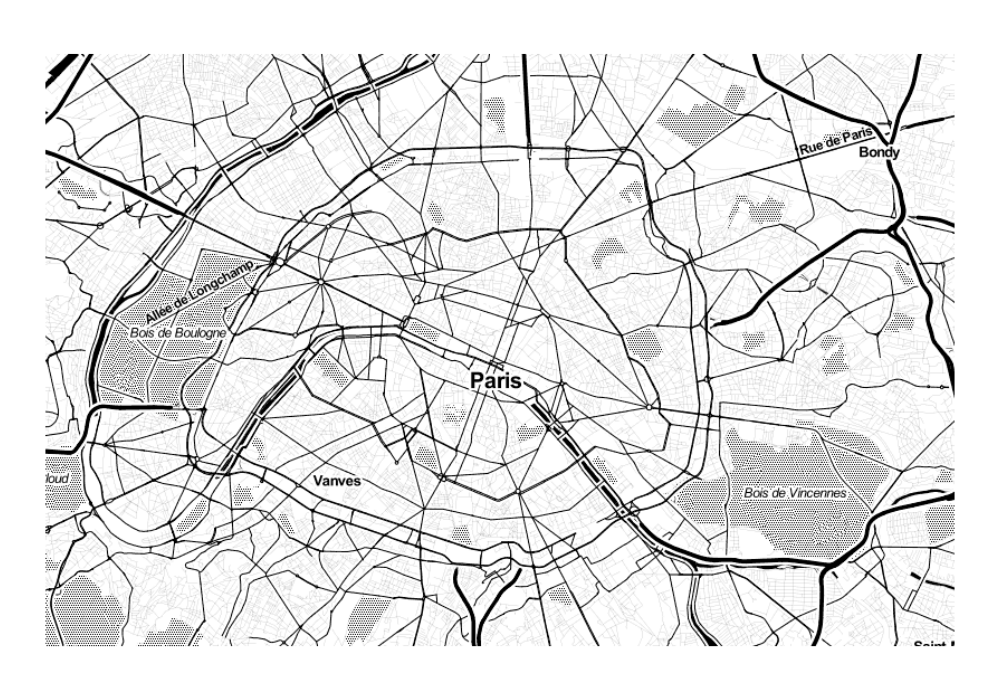# Animated bikes stocks

• A problem of balance !
• A regulation challenge
• A move // a repetitive pulse

# Stocks data : vlsstat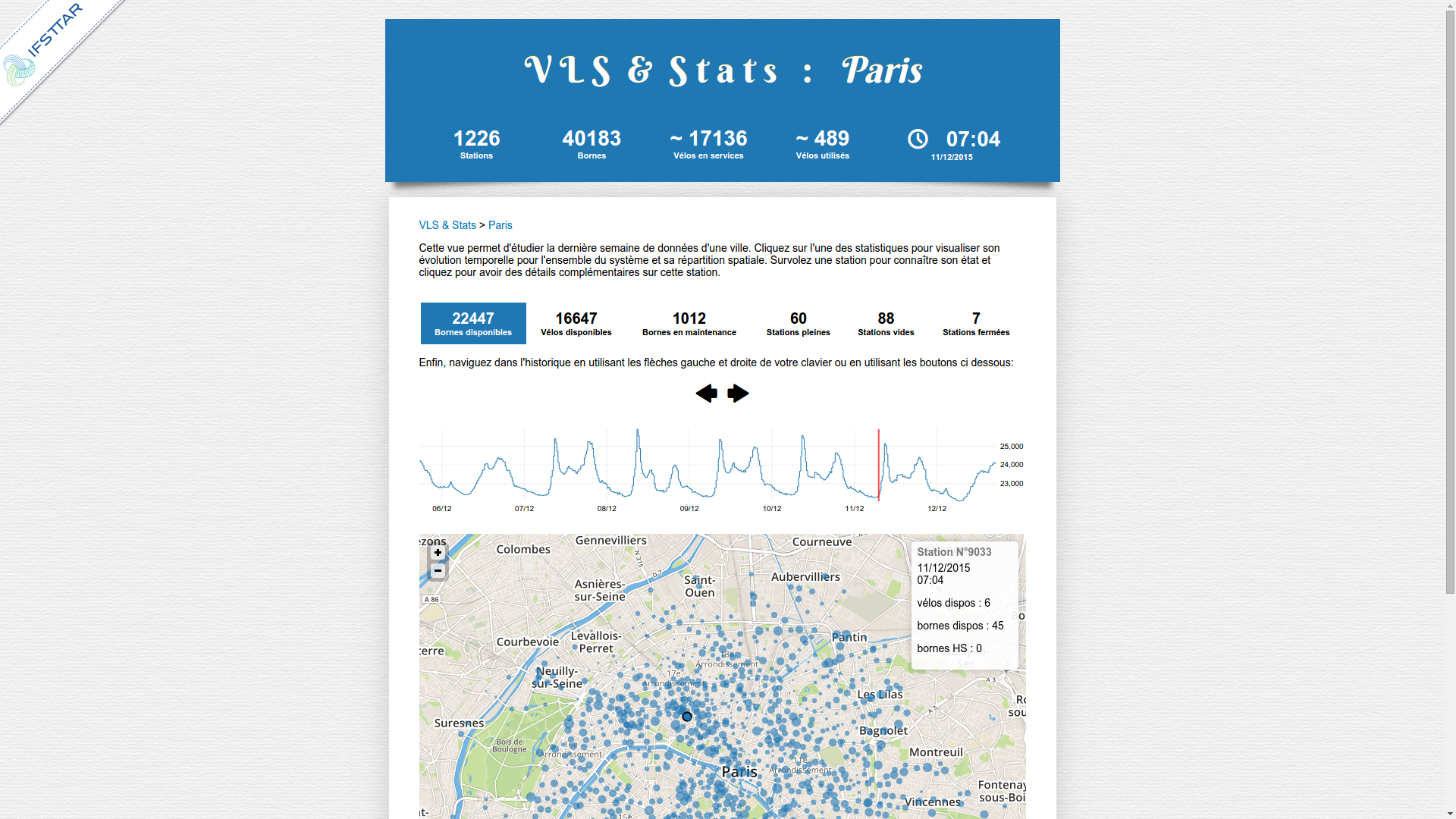# Discriminative Functional Mixture Model

\begin{eqnarray} X(t)=\sum_{j=1}^{p}\gamma_{j}(X)\psi_{j}(t),\label{eq:X} \end{eqnarray} where $\gamma=(\gamma_{1}(X),...,\gamma_{p}(X))$ is a random vector in $\mathbb{R}^{p}$, \begin{eqnarray} p(\gamma)=\sum_{k=1}^{K}\pi_{k}\phi(\gamma;U\mu_{k},U^{t}\Sigma_{k}U+\Xi), \end{eqnarray} Estimation EM + (fPCA) step = FunFEM

### Clustering of stocks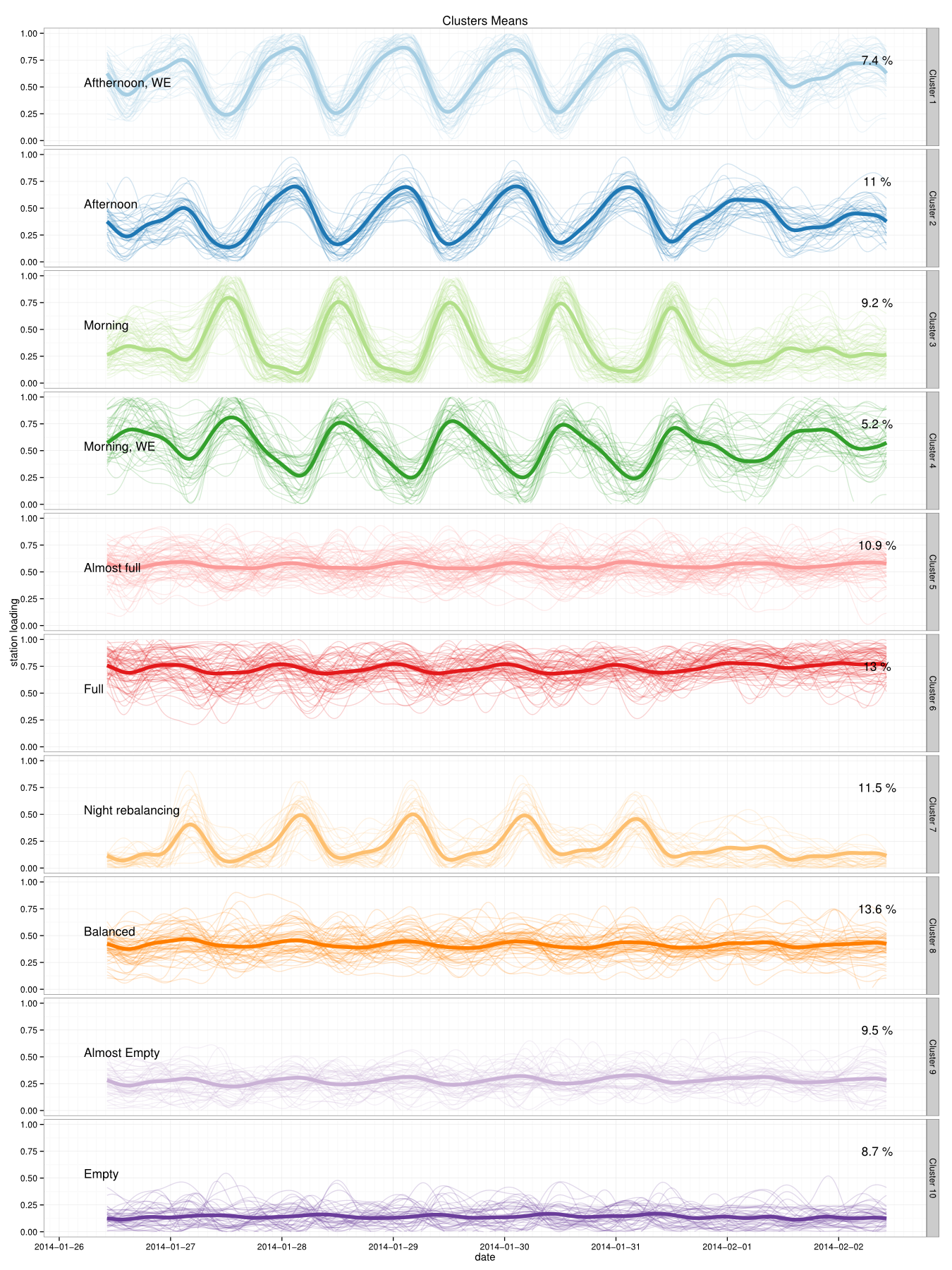The Discriminative Functional Mixture Model for the Analysis of Bike Sharing Systems [preprint]

### Clustering of stocks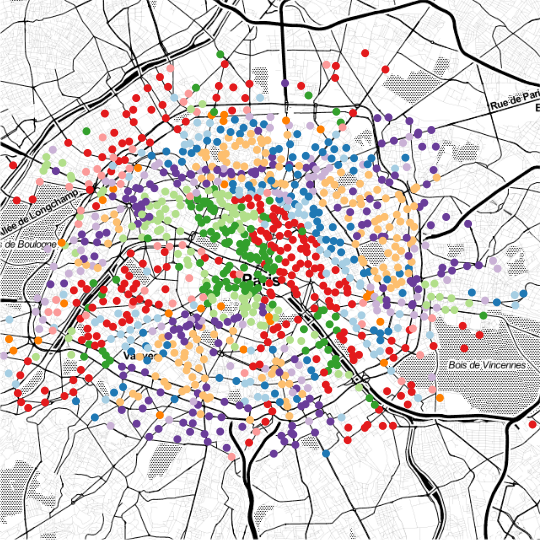The Discriminative Functional Mixture Model for the Analysis of Bike Sharing Systems [preprint]

### Clustering fonctionel sur données de stocks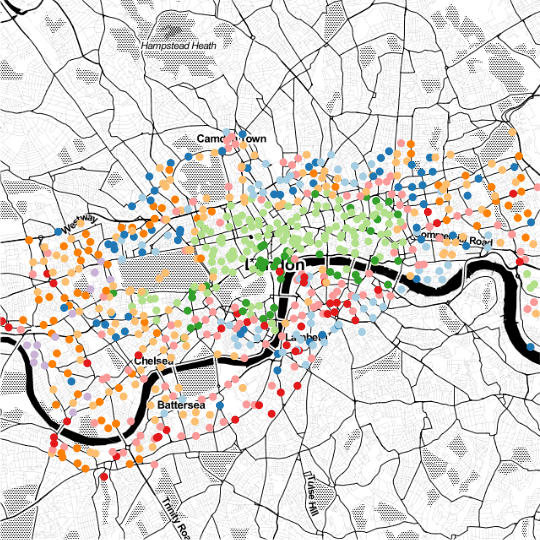The Discriminative Functional Mixture Model for the Analysis of Bike Sharing Systems [preprint]

# Model

• $X_{sdt}$ (observed): number of bikes comming/leaving
• $Z_s$ (latente): station s cluster
• $W_d$ (observed): (week / week end)

## Simple generative model :

$$Z_s\sim\mathcal{M}(1,\pi)$$ $$X_{sdt}|\{Z_{sk}=1,W_{dl}=1\}\sim\mathcal{P}(\alpha_s\lambda_{klt})$$ + constraints $\sum_{l,t}D_l\lambda_{klt}=DT, \forall k \in\{1,...,K\}$,
with $D_l$ number of days in $l$.

# Modèle

• $X_{sdt}$ (observée): number of bikes comming/leaving
• $Z_s$ (latente): station s cluster
• $W_d$ (observed): (week / week end)

## Likelihood :

$$Lc(\mathbf{\Theta};\mathbf{X},\mathbf{Z},\mathbf{\alpha},\mathbf{W})=\sum_{s,k}Z_{sk}\log\left(\pi_{k}\prod_{d,t,l}po(X_{sdt};\alpha_s\lambda_{klt})^{W_{dl}}\right)$$ Estimation EM, + extension to use meteo data

# Urban dynmics through the observed flows

• An interesting viewpoint
• Easy interpretation
• Crossing with contextual data
• ...### Crossing with contextual data // socio-eco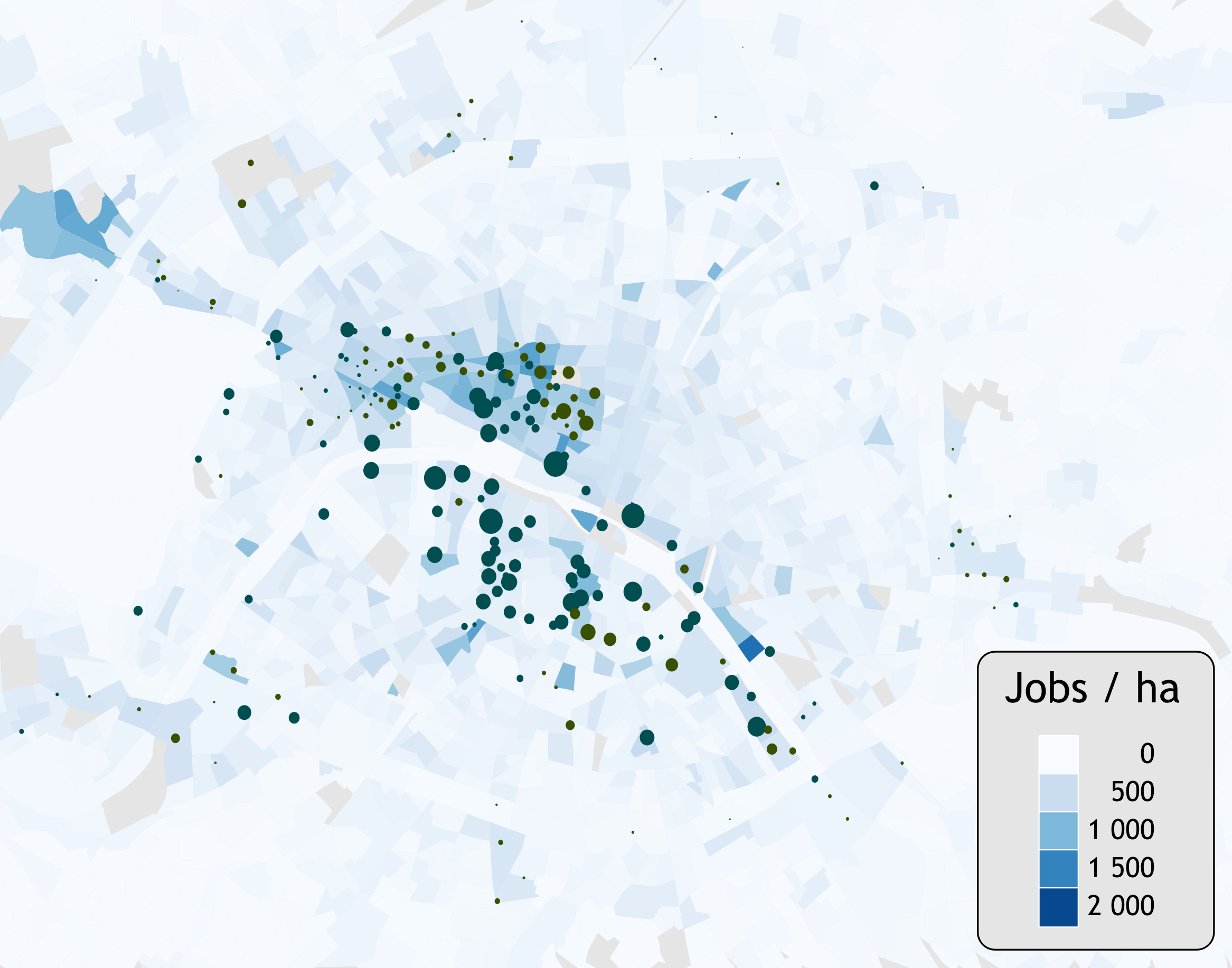### Crossing with contextual data // socio-eco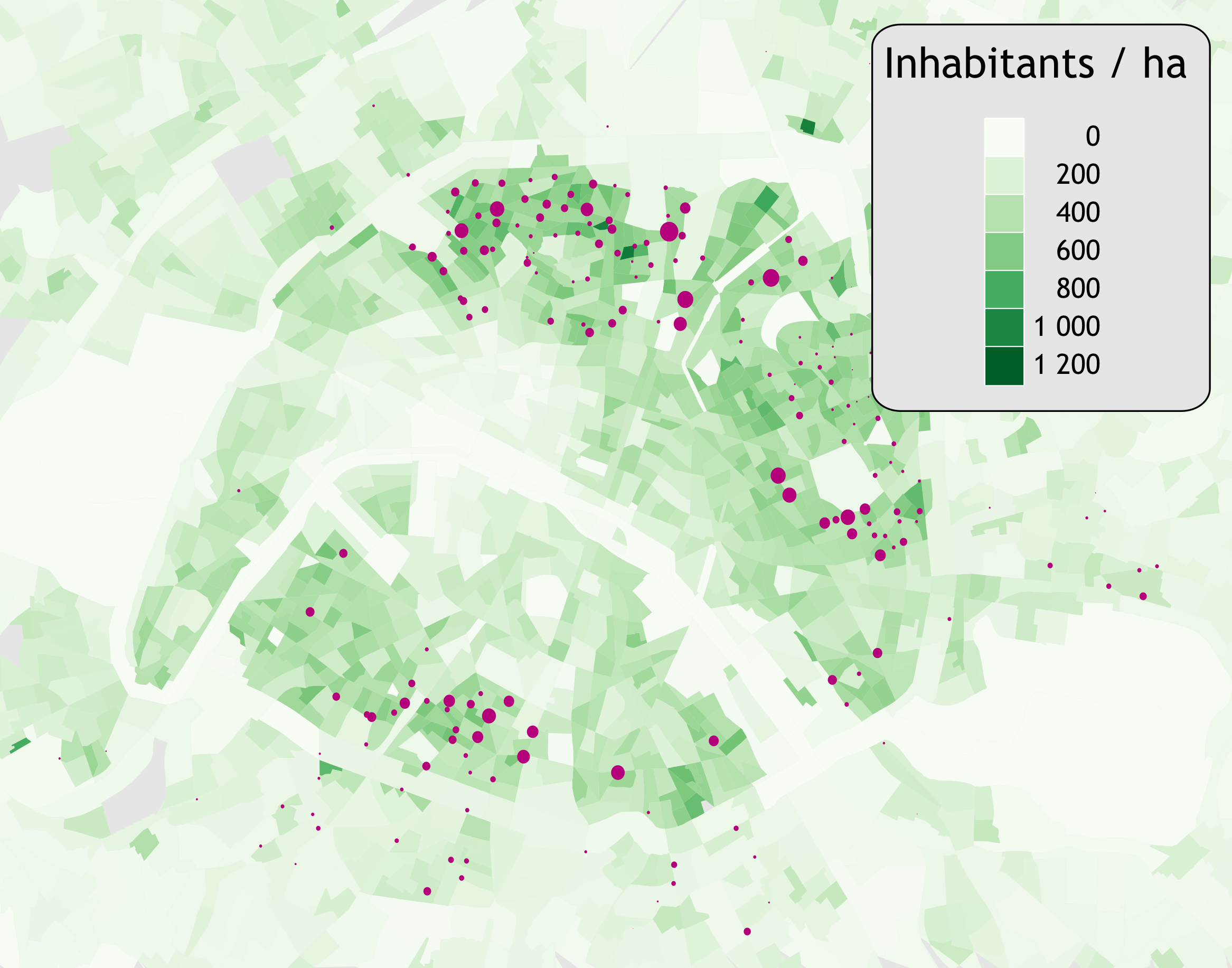### Crossing with contextual data // socio-eco

 hab/ha emp/ha serv/ha com/ha * 162 237 4.2 3.7 Leisure (1) 367 189 6.3 4.4 Leisure (2) 261 322 7.7 6.9 Parks 172 90 2 1.7 Stations 209 206 2.4 1.8 375 108 3.8 2.7 Jobs(1) 138 409 4.5 2.8 Jobs(2) 157 456 5.7 5.6 Average 301 163 3.8 2.8

# Latent Dirichlet Allocation

## for dynamical O/D matrices analysisLocal stationarity of BSS behaviour / OD Small bags of successive trips $\approx$ stationarity of OD
Documents (bags of words) = bags of successive trips (5000)
With :
• Words = Origin/Destination couples
• Topics = Latent activities

# Latent Dirichlet Allocation

#### For dynamic Origine-Destination matrices analysis

For each latent activity$a$ , draw its template : $\Lambda_a\sim\mathcal{D}(\beta)$
For each bag of trips
Draw the proportion of activities : $\pi_t \sim \mathcal{D}(\alpha)$
For each trips
Draw its activity
$A \sim \mathcal{M}(1,\pi_t)$
Draw an OD using the activity template
$D \sim \mathcal{M}(1,\Lambda_A)$

# OD (Tensor) decomposition resultsModel selection with perplexity analysis
(clear drop for K=5)

# Latent activity template $\Lambda_a$

Draw Nt, (number of trips) using $\Lambda_a$ : $$OD\sim\mathcal{M}(Ndep,\Lambda_a)$$ Compute the balance (incoming bikes - leaving bikes) for a station $s$ : $$B_s=\sum_jOD_{js}-\sum_jOD_{sj}$$ Compute the expectation for each station $\mathbb{E}[\mathbf{B}]=Ndep(\Lambda_a^t-\Lambda_a)\mathbf{1}$

# Stations Balances : home→work# Stations Balances: work→home# Stations Balances : begining of evening# Gravity-LDA

#### LDA extension for taking stations context into-accounts

Replace the O/D matrix templates $\Lambda_k$ by a parametric form which depends on :
• context of departure station, $\mathbf{x_u}$
• context of arrival station, $\mathbf{x_v}$
• distance between $u$ and $v$, $\mathbf{x_{uv}^{da}}$
\begin{equation} \Lambda_{\,uv}(\Theta_k) \,=\, \frac{ \exp(\mathbf{\theta_{k}^{d}}^\top \mathbf{x_{u}} + \mathbf{\theta_{k}^{a}}^\top \mathbf{x_{v}} + \mathbf{\theta_{k}^{da}}^\top \mathbf{x_{uv}^{da}}) } {\sum\limits _{u,v}\; \exp(\mathbf{\theta_{k}^{d}}^\top \mathbf{x_{u}} + \mathbf{\theta_{k}^{a}}^\top \mathbf{x_{v}} + \mathbf{\theta_{k}^{da}}^\top \mathbf{x_{uv}^{da}}) } \end{equation}

# Gravity-LDA

#### LDA extension for taking stations context into-accounts

Replace the O/D matrix templates $\Lambda_k$ by a parametric form which depends on :
• context of departure station, $\mathbf{x_u}$
• context of arrival station, $\mathbf{x_v}$
• distance between $u$ and $v$, $\mathbf{x_{uv}^{da}}$
\begin{equation} \Lambda_{\,uv}(\Theta_k) \,=\, \frac{ \exp(\mathbf{\theta_{k}^{d}}^\top \mathbf{x_{u}} + \mathbf{\theta_{k}^{a}}^\top \mathbf{x_{v}} + \mathbf{\theta_{k}^{da}}^\top \mathbf{x_{uv}^{da}}) } {\sum\limits _{u,v}\; \exp(\mathbf{\theta_{k}^{d}}^\top \mathbf{x_{u}} + \mathbf{\theta_{k}^{a}}^\top \mathbf{x_{v}} + \mathbf{\theta_{k}^{da}}^\top \mathbf{x_{uv}^{da}}) } \end{equation}

# Conclusion on OD analysis

• Easy segmentation of time
• Compact model of the system few template
• Evidences of clear cycles
• Also used for other type of data Bluetooth, smartcard,....
• Can be interesting for simulation
• Only satified demand is taken into account (users that do not find bikes!)

#### Extension

• Socio-economic factors + od factors (distances, type of roads) can be integrated
$\Rightarrow$ Usefull for new station planning (system extension)

# Bonus : cities portraits at Night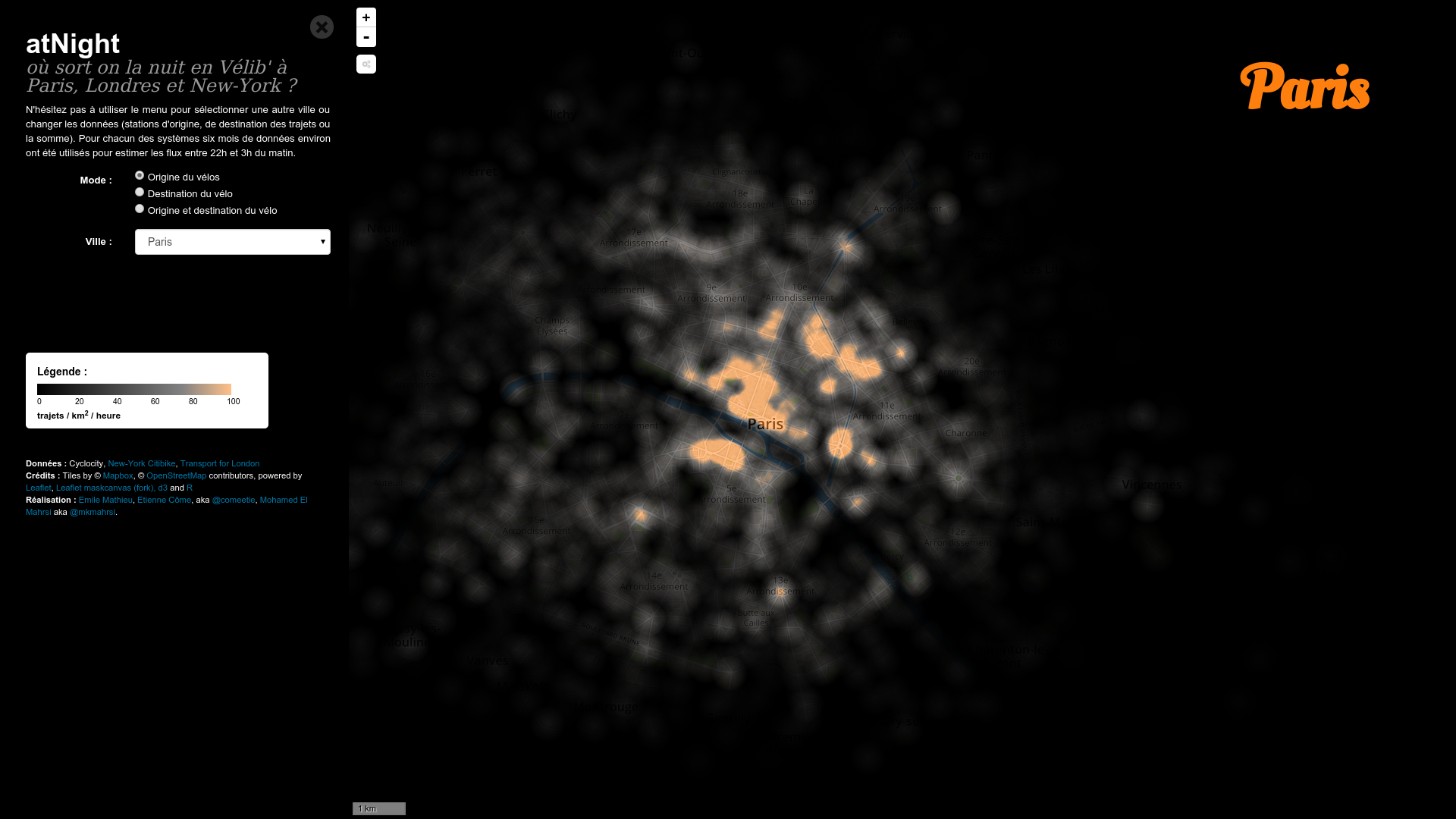# Bonus : cities portraits : atNight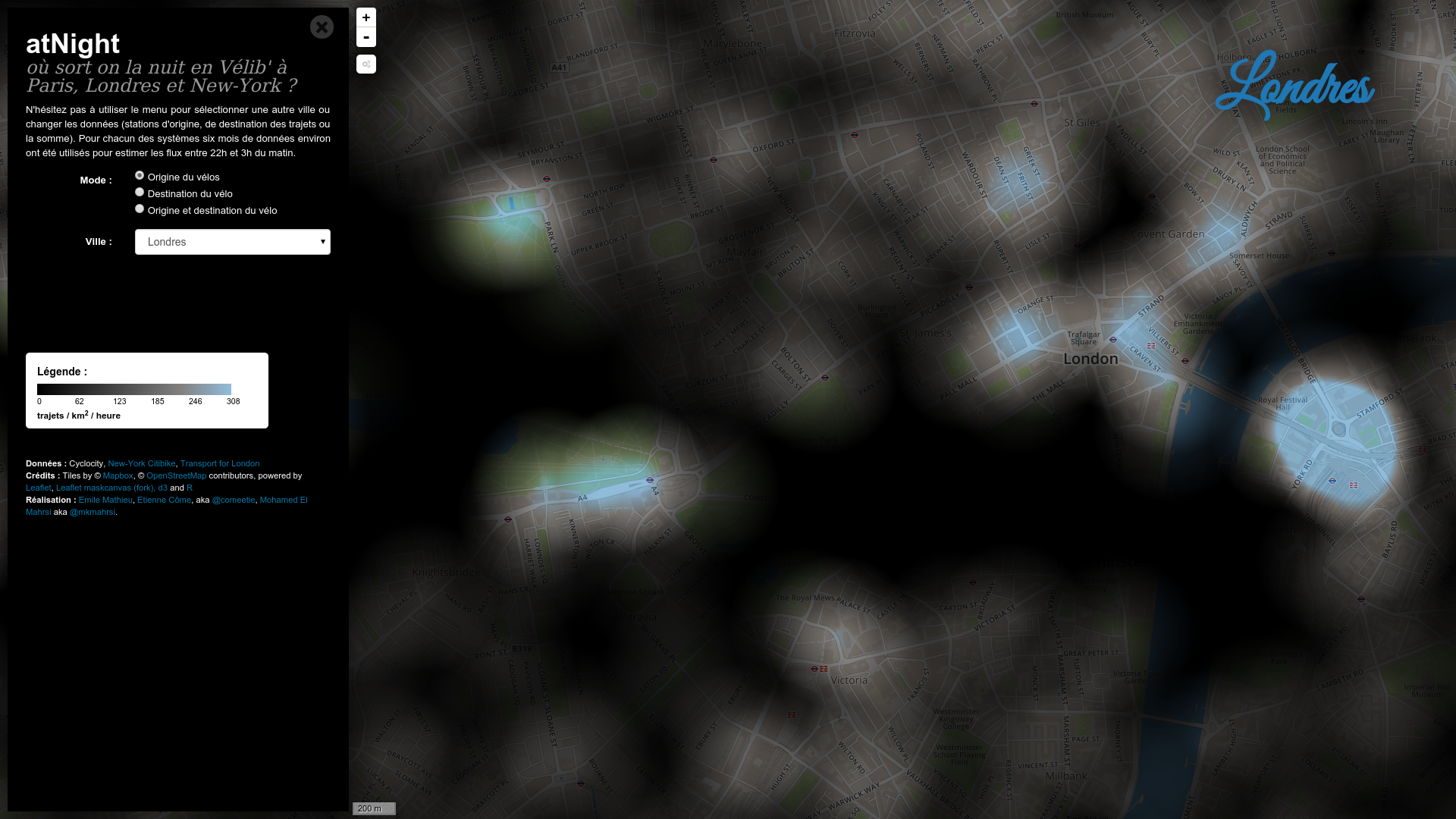# Transit networks analysis# Smart-card data form

• anonymous user id (changed every 3 month)
• type of card
• stop id (+line and direction for the bus)
• date and time

#### Open-Data ? (ex: "Ile de France Mobilité" in aggregated form)# Smart-card data form

• anonymous user id (changed every 3 month)
• type of card
• stop id (+line and direction for the bus)
• date and time

#### A particular field : user id# A massive dataset

• anonymous user id (changed every 3 month)
• type of card
• stop id (+line and direction for the bus)
• date and time

#### With user ids ?

• Analyzing in-flow volumes
• Days clustering
→ outliers detection, mid term prediction,...
• Stations clustering
→ spatial analysis of the demand, ...

#### Without user ids ?

• Destination inference
→ transfers analysis, dynamical OD matrices,...
• Short term OD matrix prediction
• Users clustering

# Analyzing in-flow volumes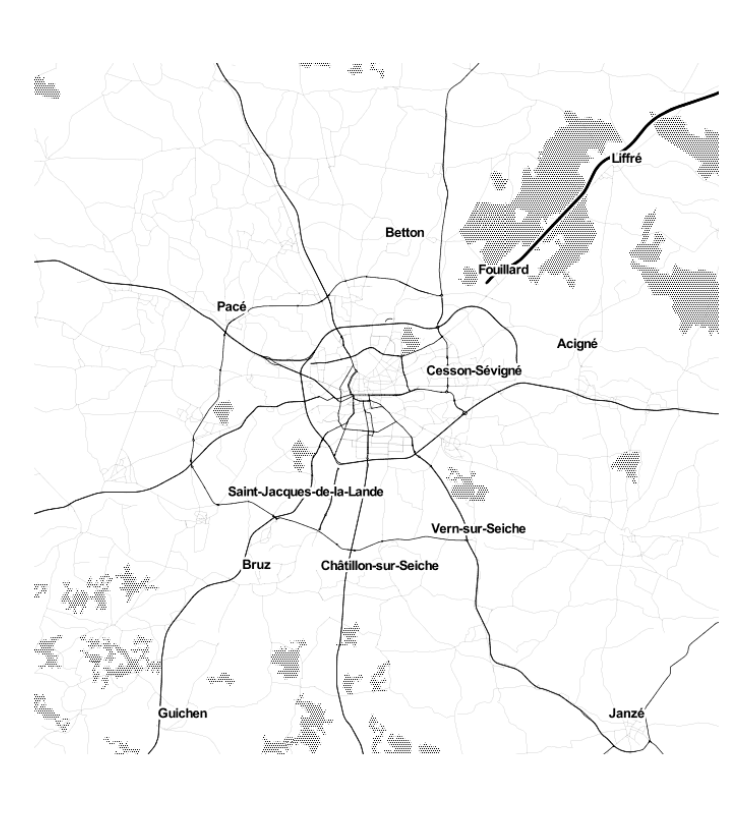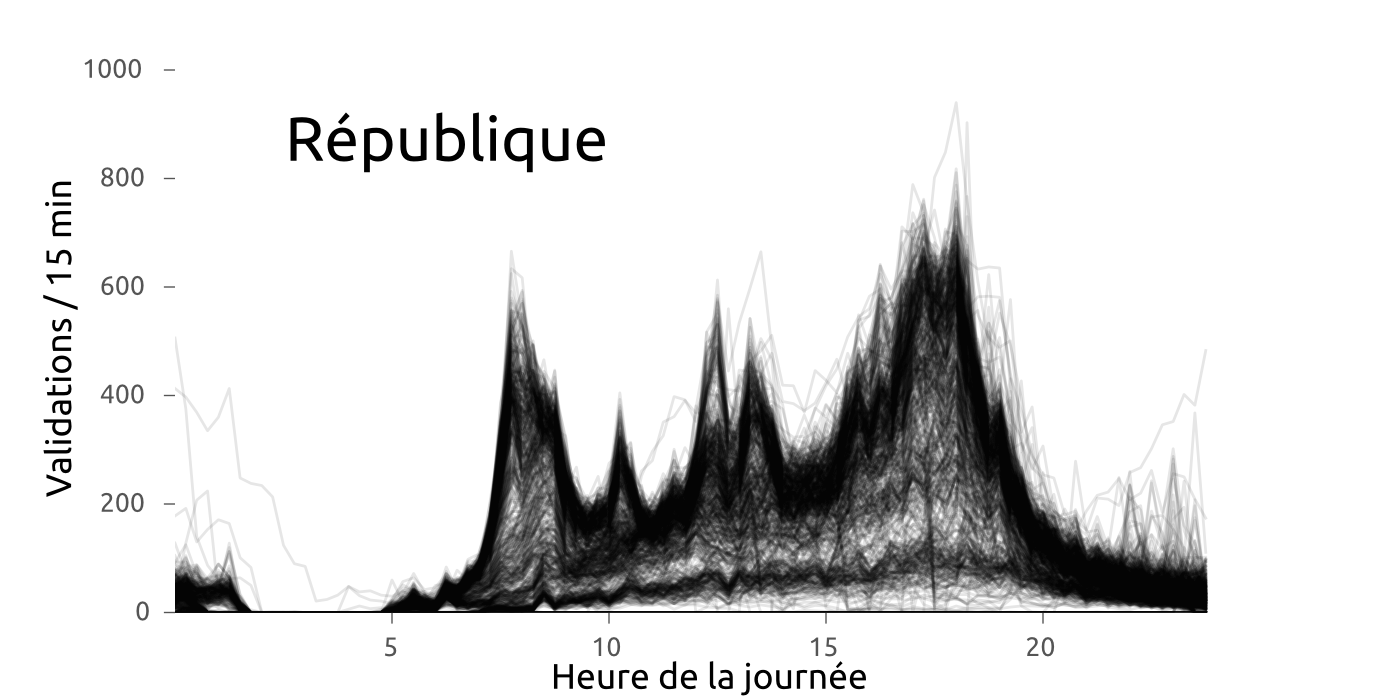#### Profiles of the demand with spatio-temporal variations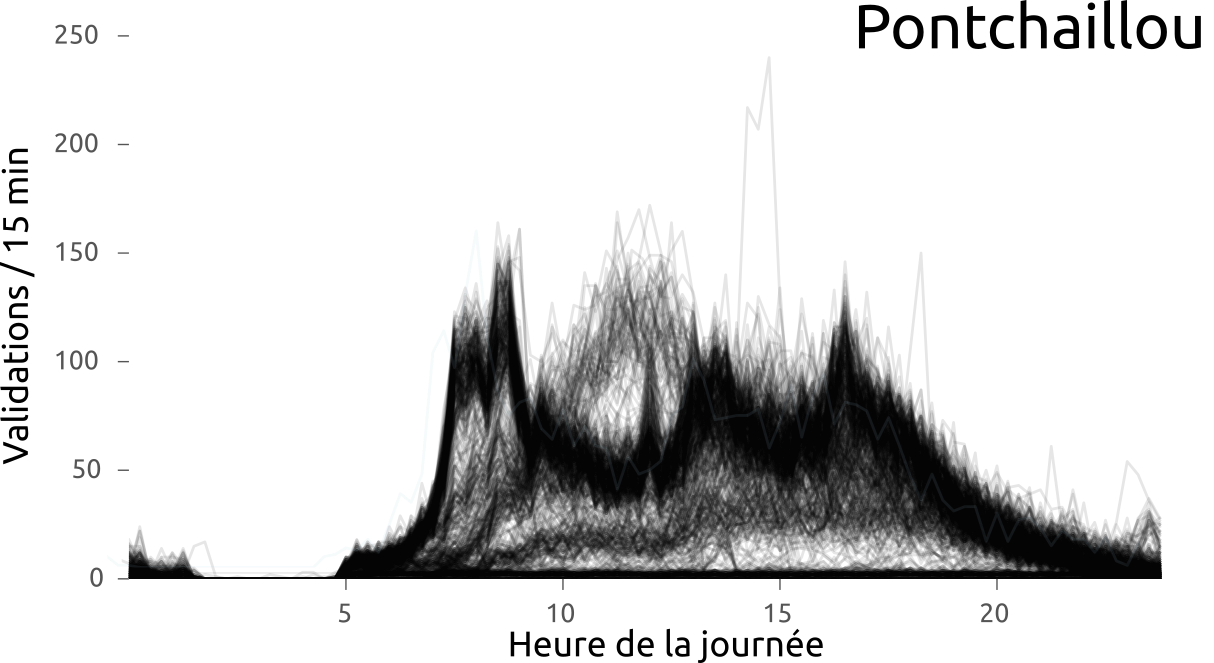#### Profiles of the demand with spatio-temporal variations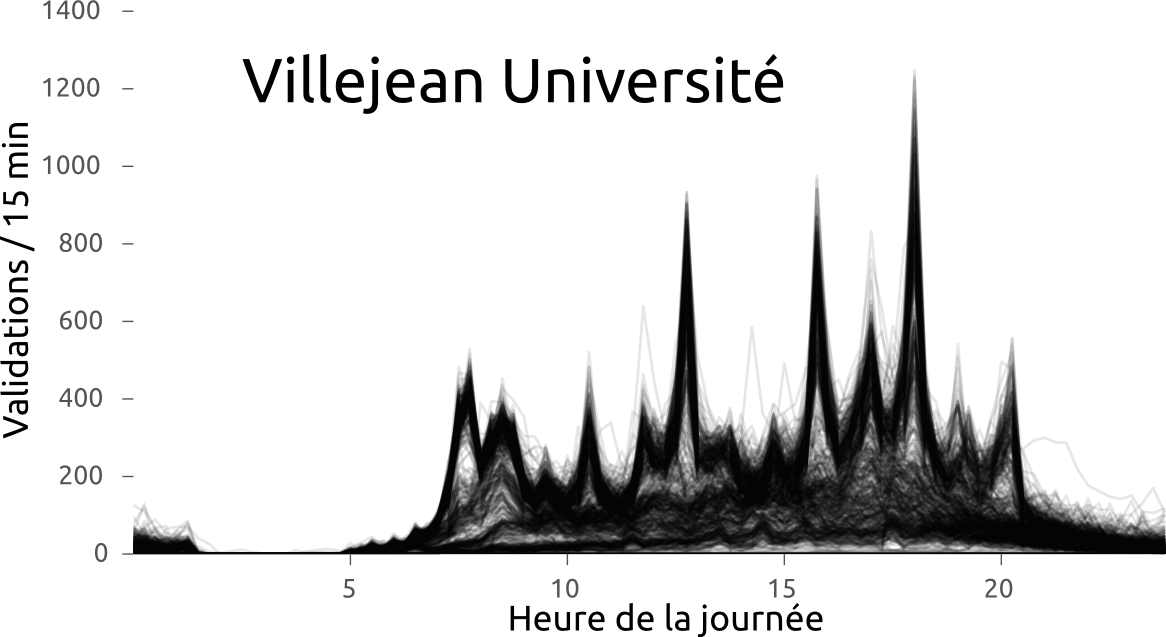### Between day variations clearly visible (CAH)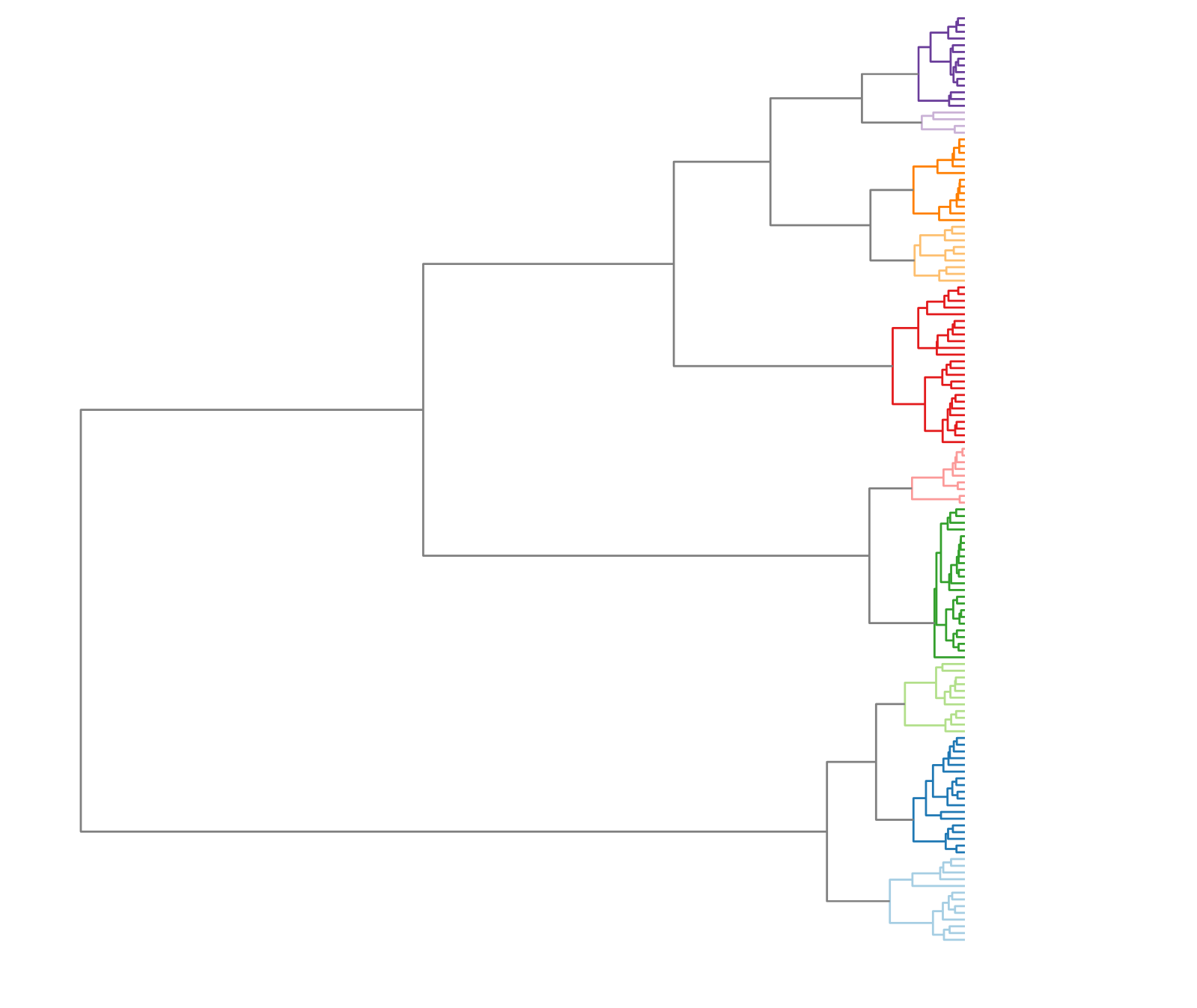### Between day variations clearly visible (CAH)### Between day variations clearly visible (CAH)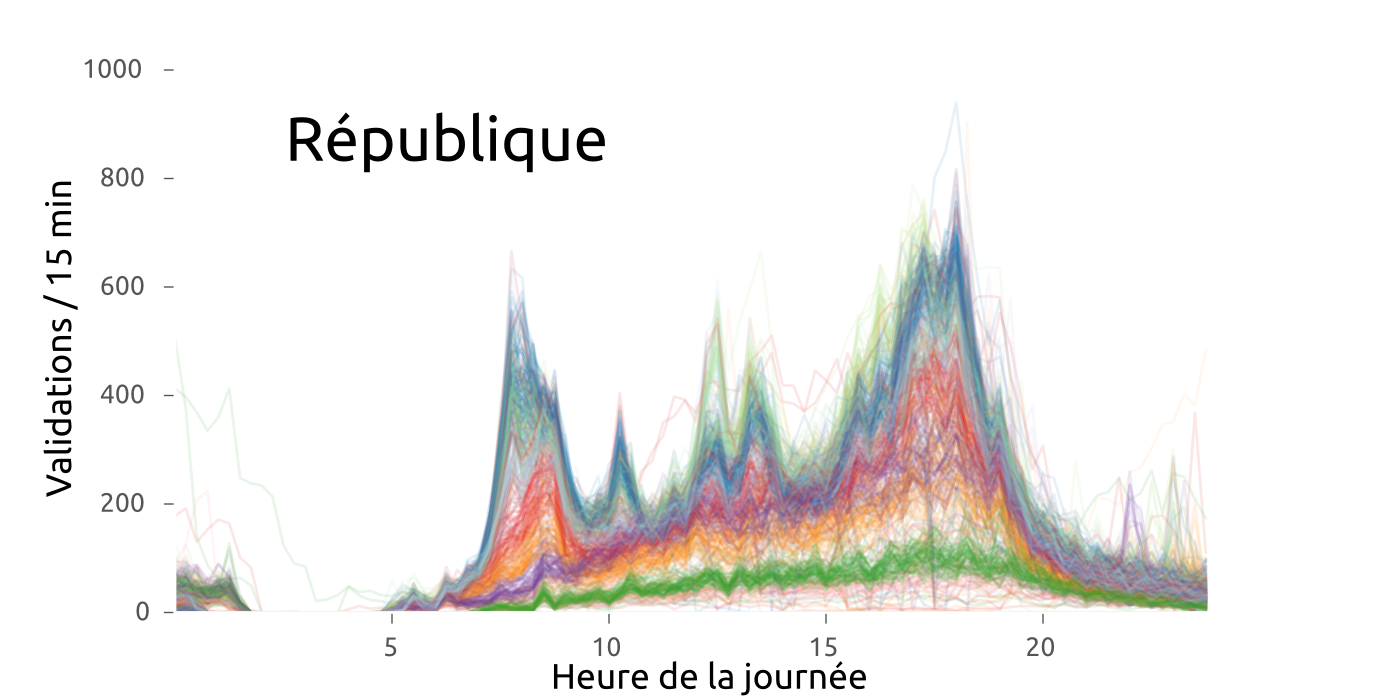### Between day variations clearly visible (CAH)### Between day variations clearly visible (CAH)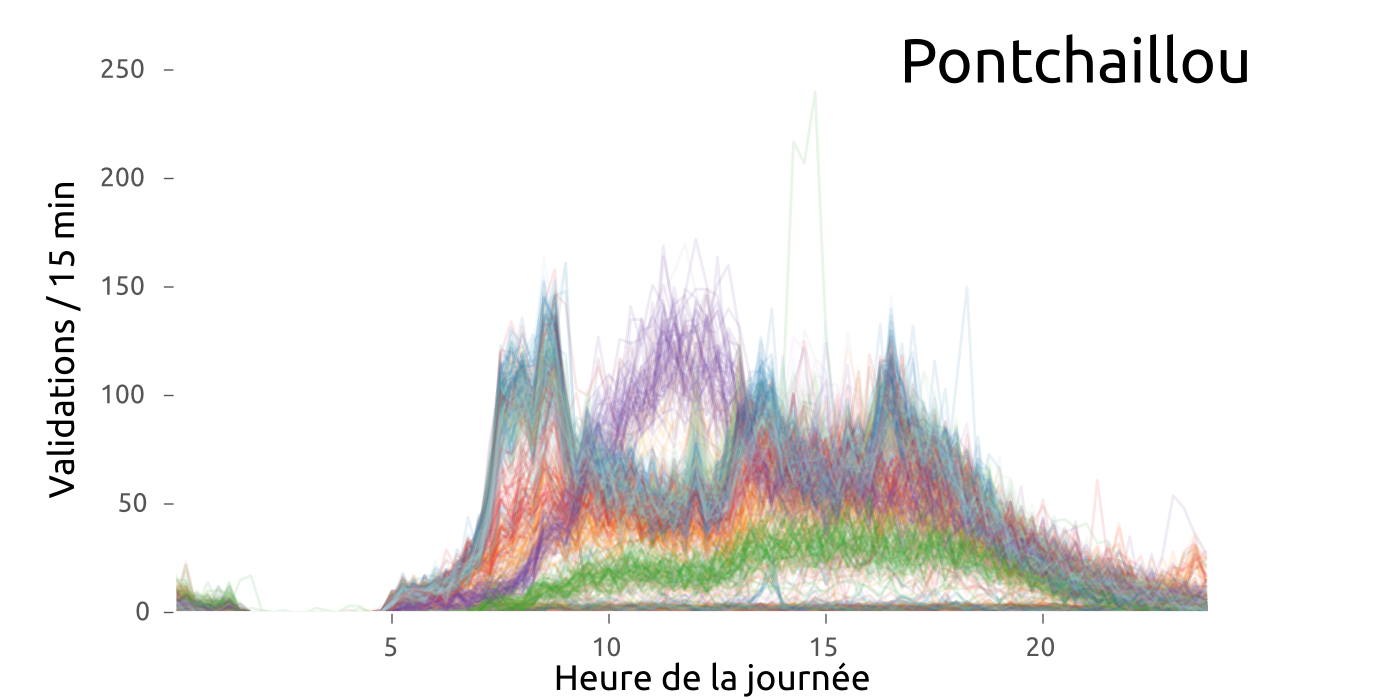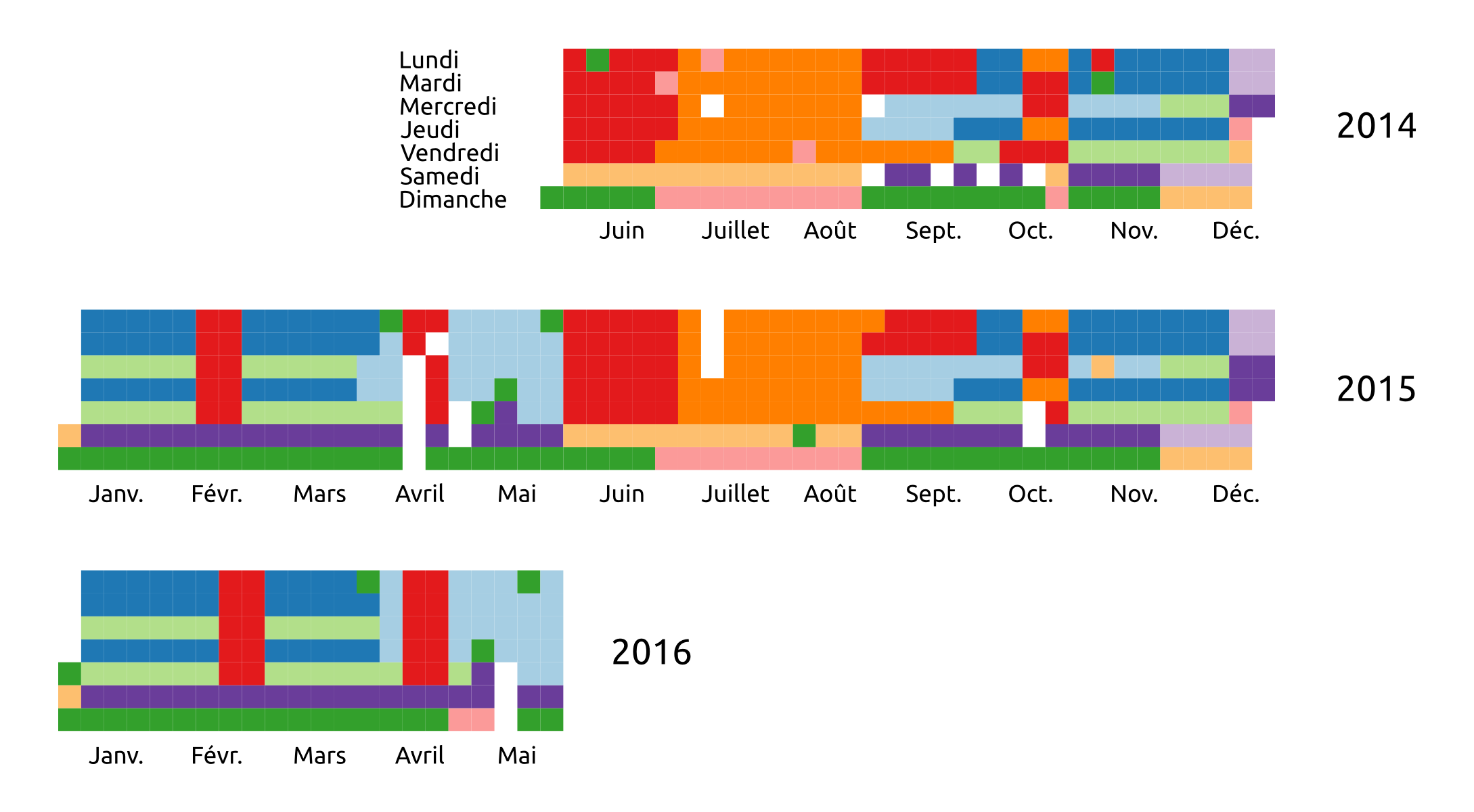#### Which is mainly explained by calendar effects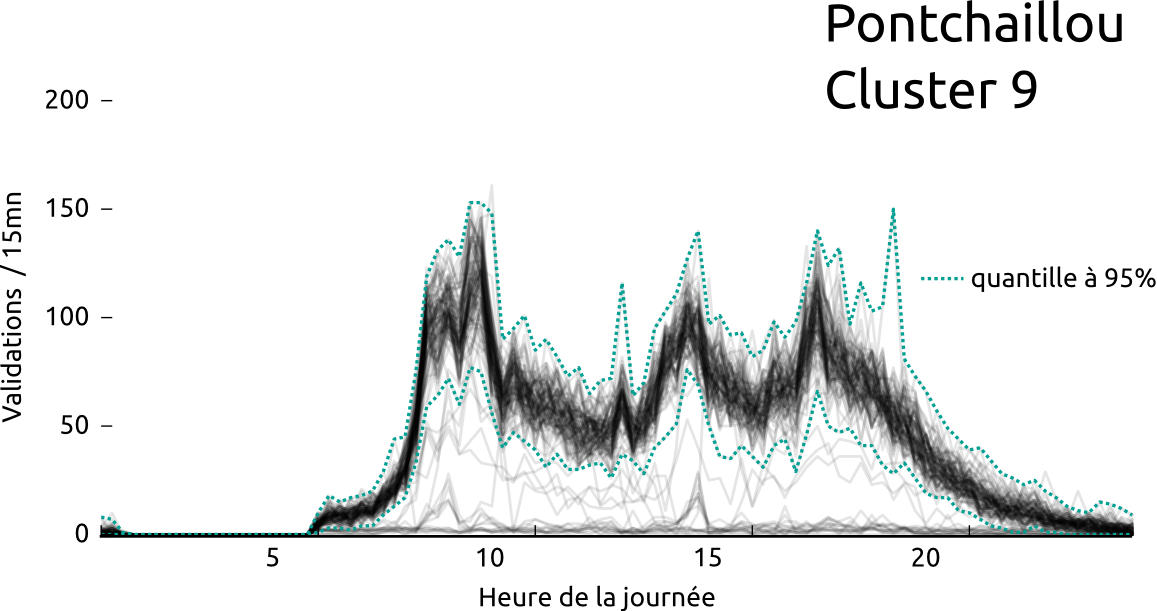#### Which can be used to detects outliers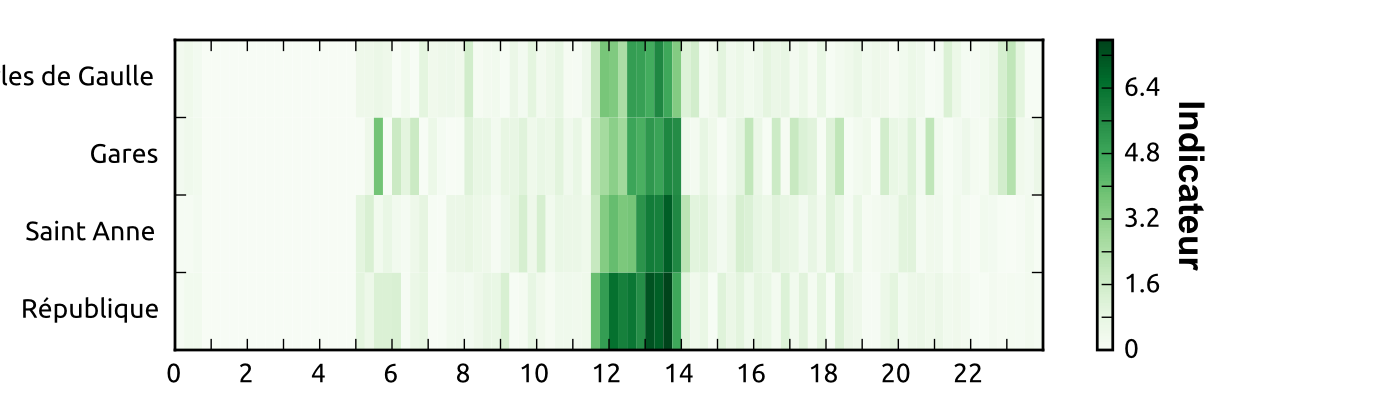#### Which can be used to detects outliers

#Rennes #metro #Star des chaises jetées sur la ligne aérienne de métro à Villejean. Dégâts importants. Trafic interrompu pendant 2h?

— Samuel Nohra (@SamuelNohra) 29 mars 2016#### Or perform mid-term predictions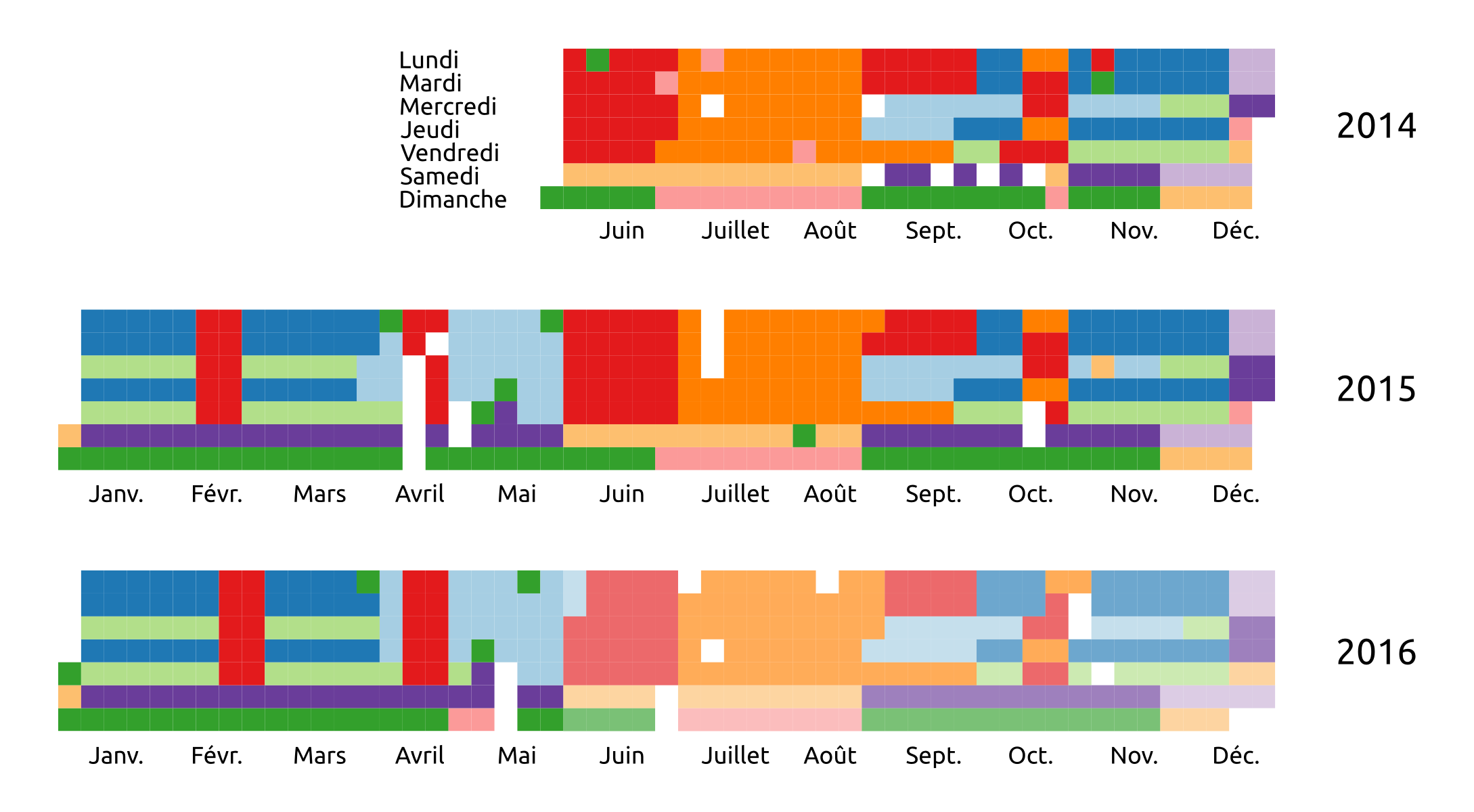# data enrichment

### Enable the reconstruction of a significant portion of the destinations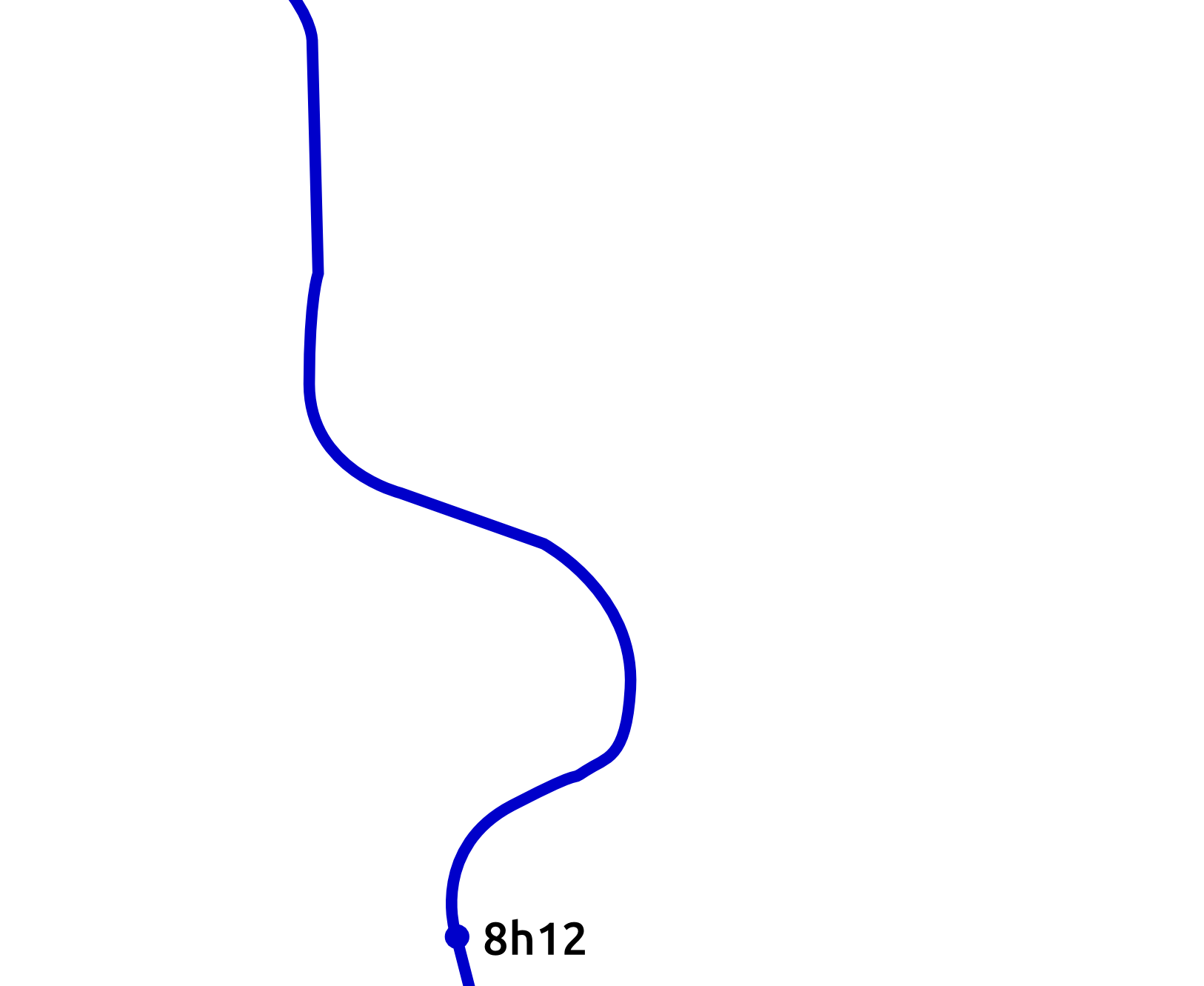### Enable the reconstruction of a significant portion of the destinations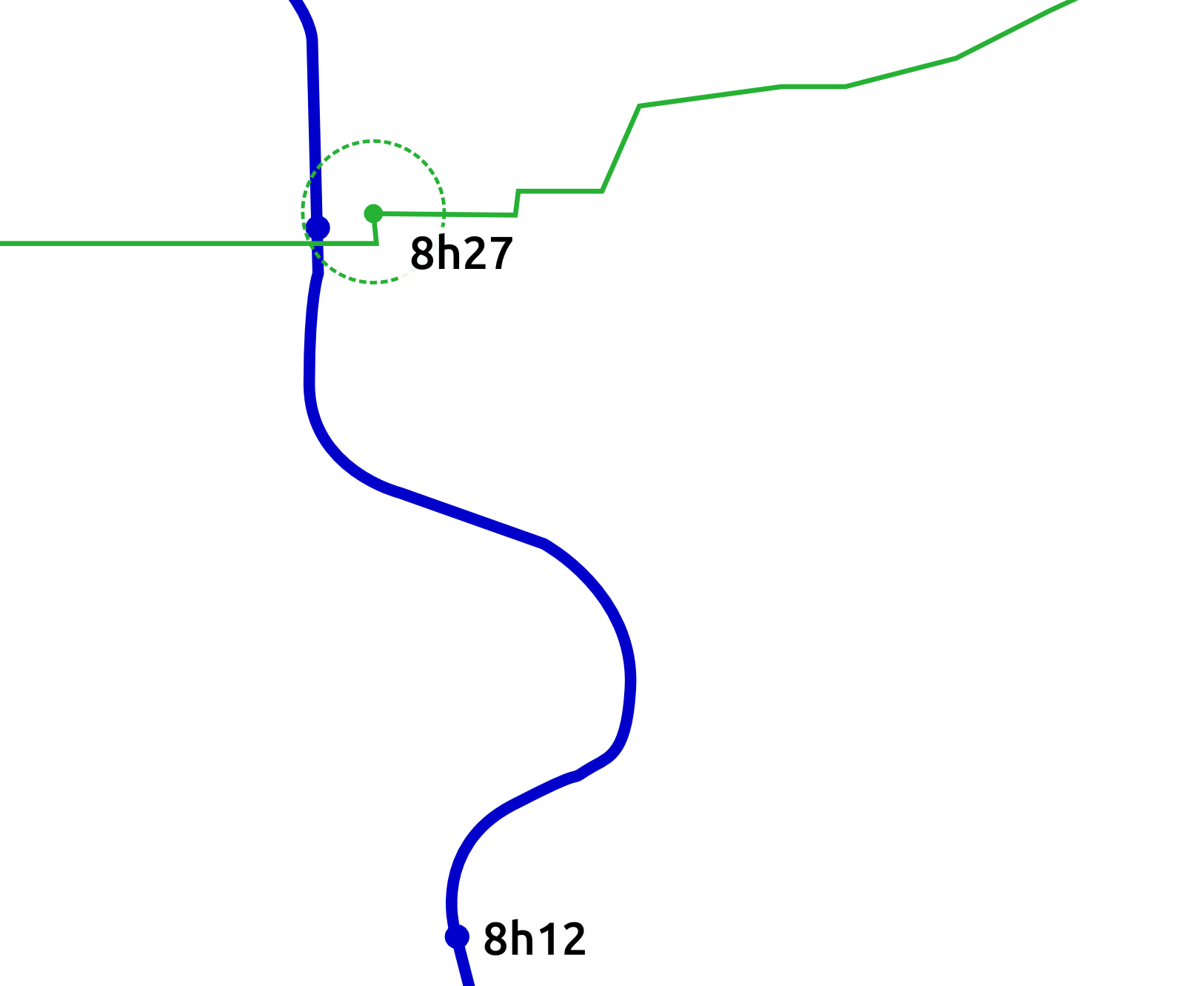### Enable the reconstruction of a significant portion of the destinations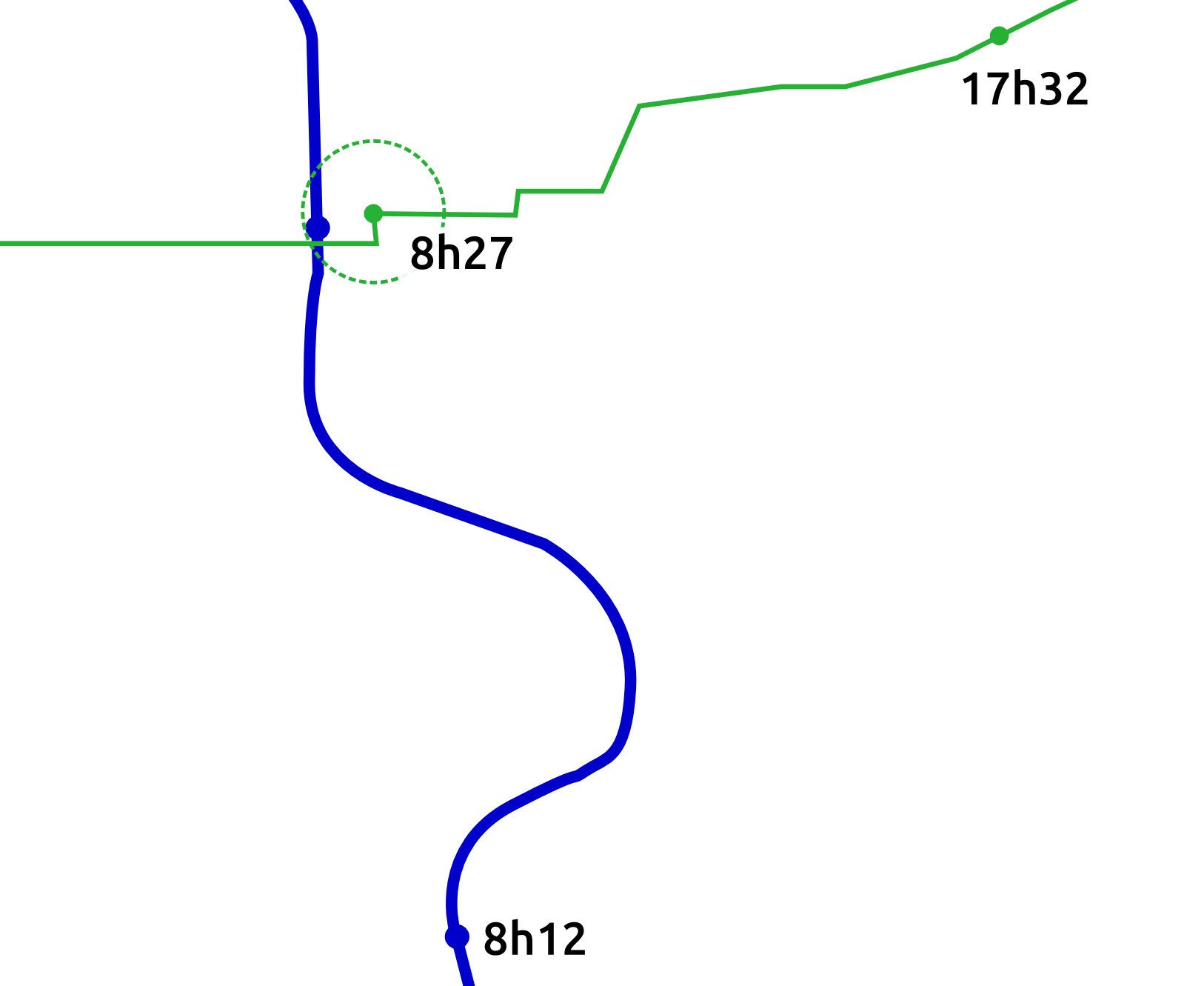### Enable the reconstruction of a significant portion of the destinations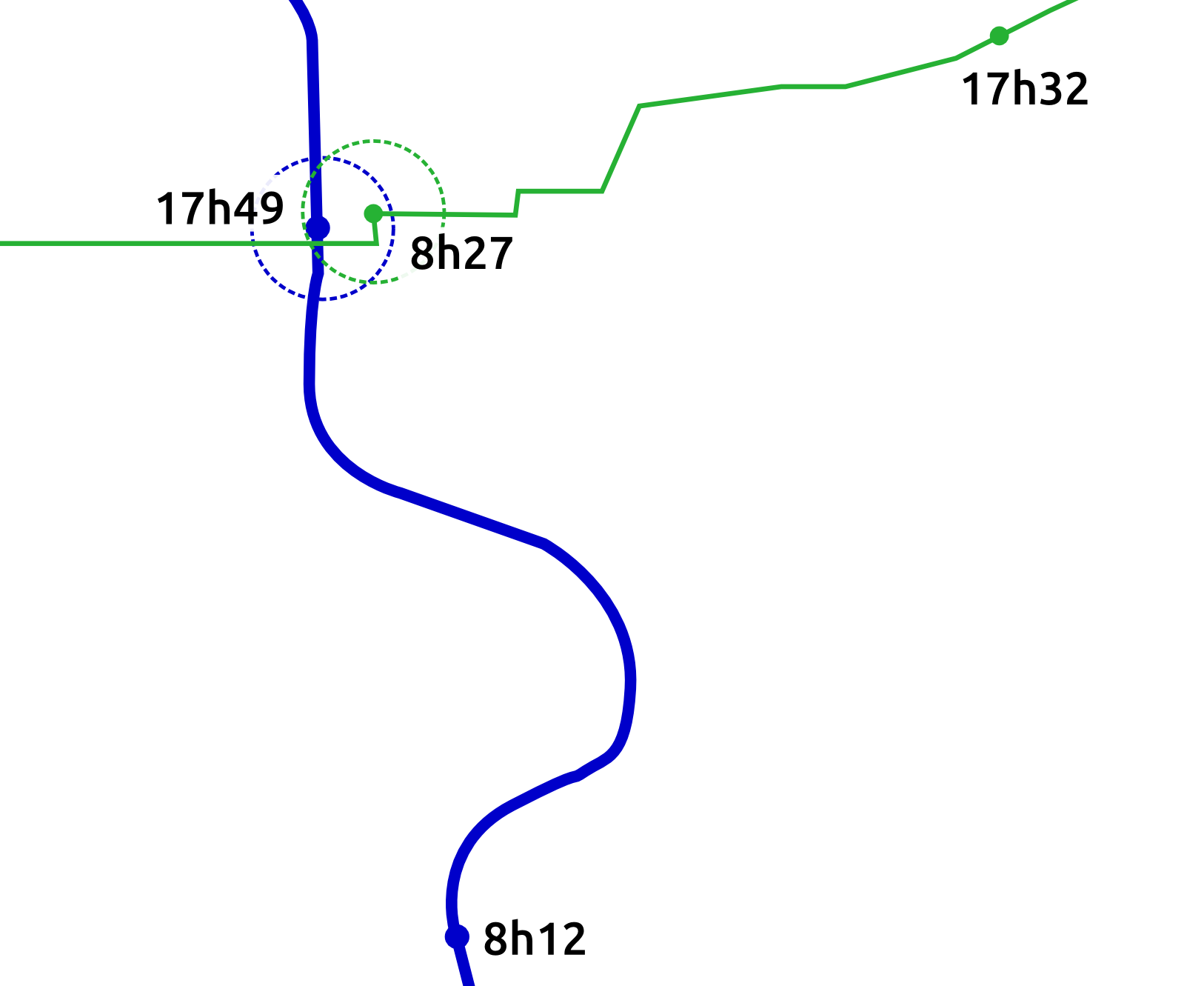### Enable the reconstruction of a significant portion of the destinations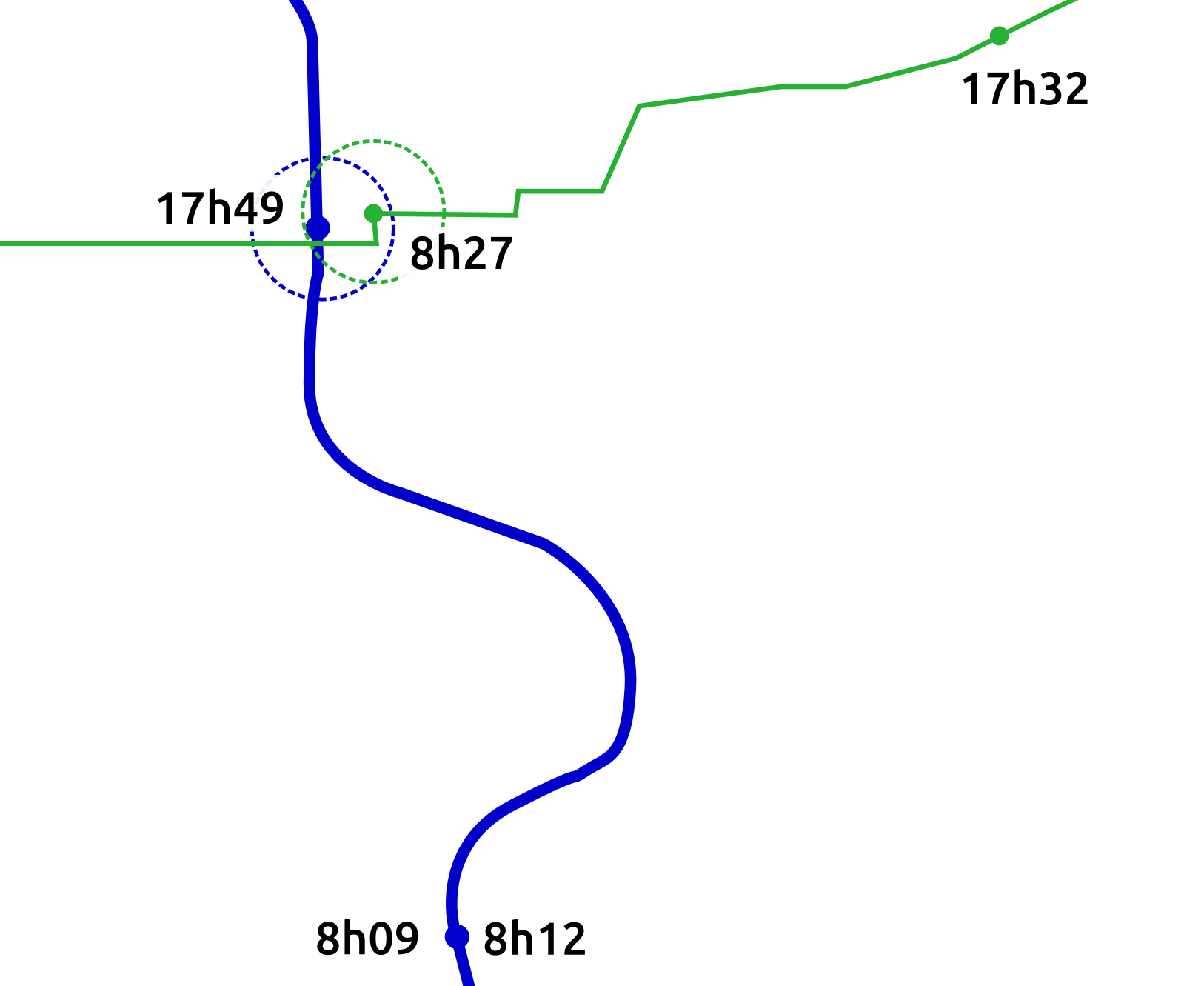# Data enrichment

• Use of next departure station to infer destination
• Temporal and spatial threshold
• Transfers detection
• User's ids must be kept 48h at minimum

### 72% of reconstructed destinations

→ Aggregation and analysis per Oirigines/Destinations
→ Multimodal exchange hub analysis (C. Richer)
→ Dynamic OD matrices or Line graph of load

# for user centric analysis

#### Objectives

• Study temporal regularity of user behaviors
• Understand how the global demand is decomposed
• Better understand users

#### Methodology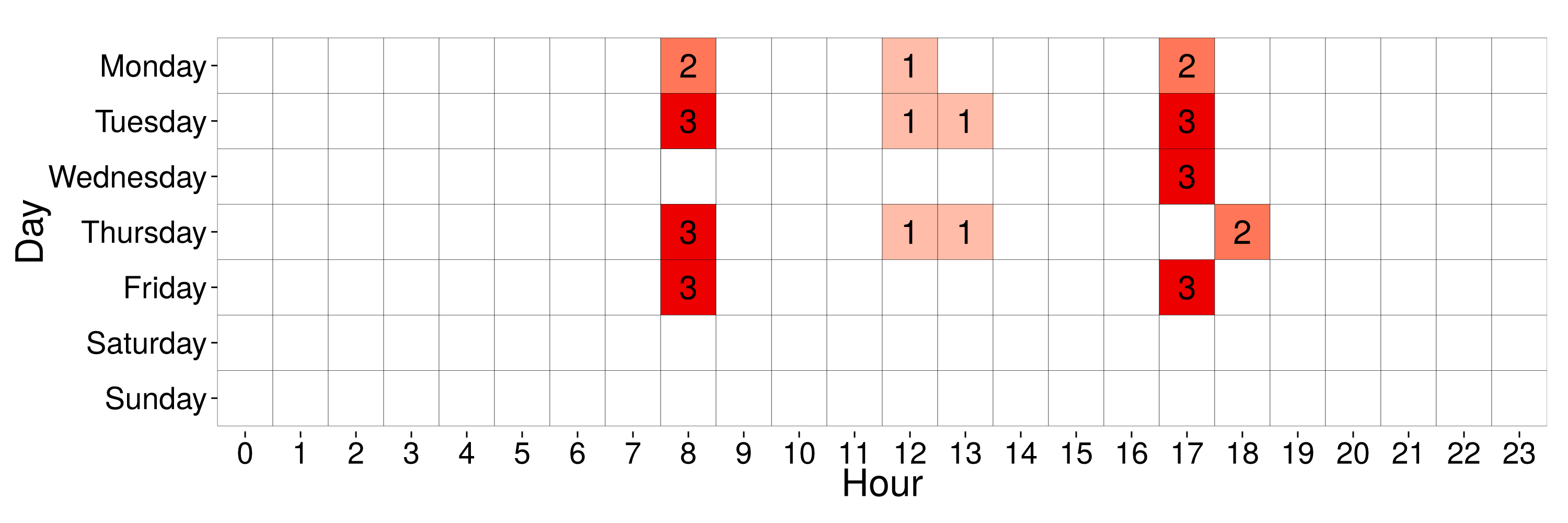• Build user profiles

#### Objectives

• Study temporal regularity of user behaviors
• Understand how the global demand is decomposed
• Better understand users

#### Methodology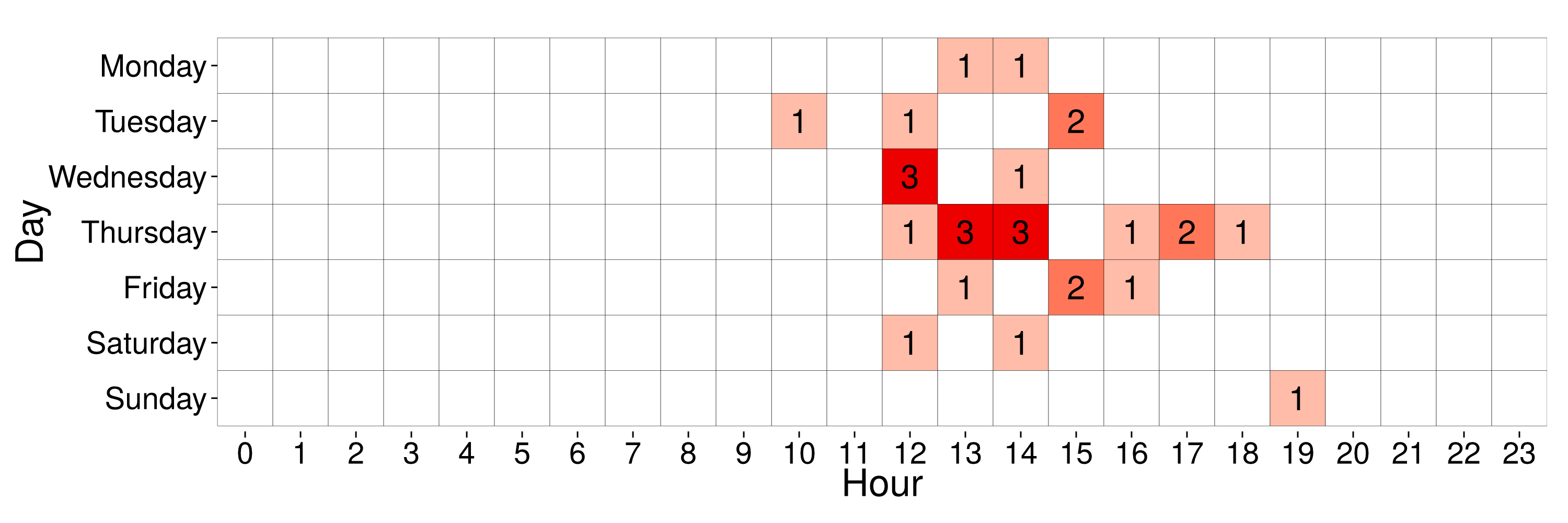• Build user profiles

# Commuter patterns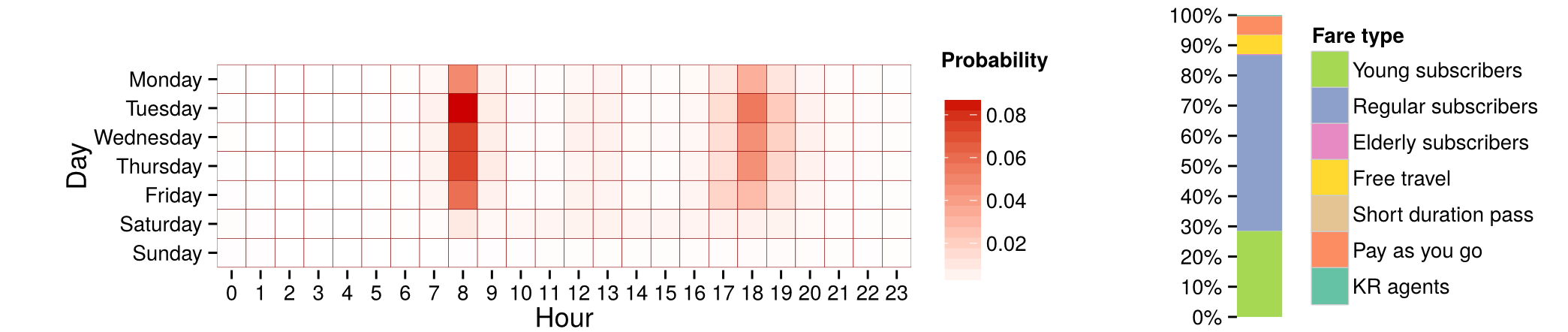Mean profile of a cluster with 4.55% of users

# Commuter patterns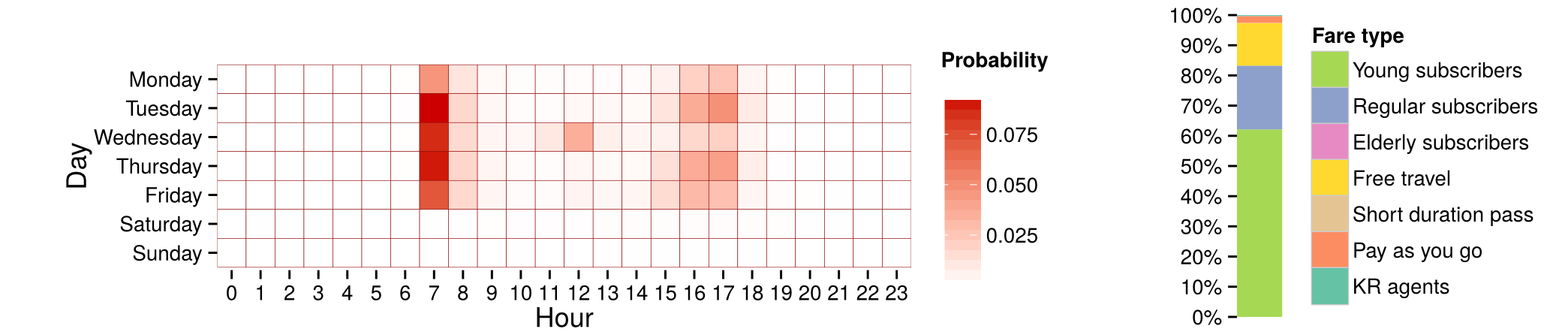Mean profile of a cluster with 12.54% of users

# Commuter patterns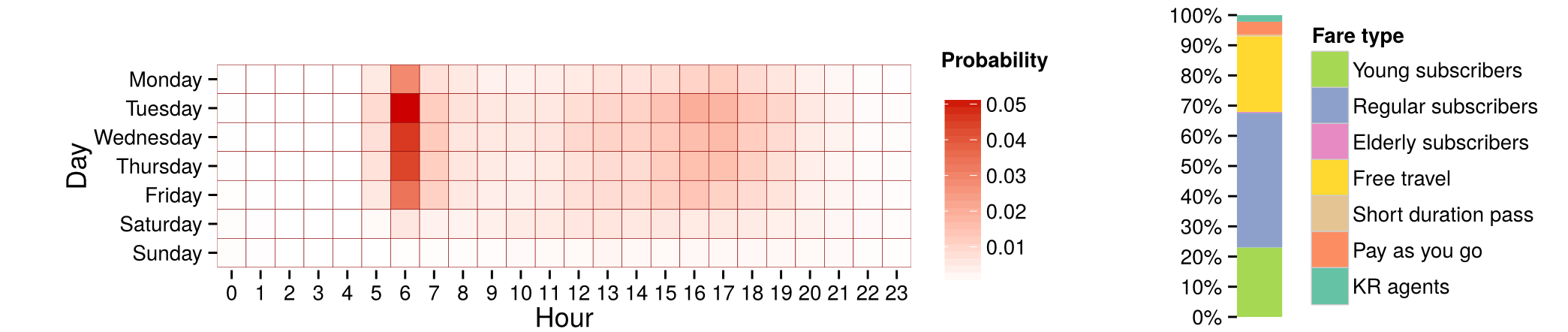Mean profile of a cluster with 3.6% of users

# But other forms emergeMean profile of a cluster with 15.13% of users

# But other forms emerge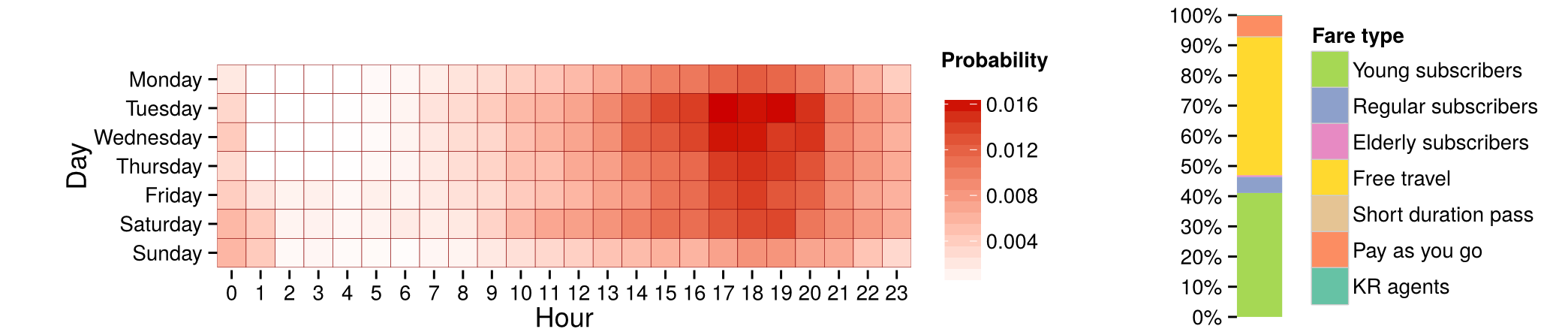Mean profile of a cluster with 6.44% of users

# But other forms emerge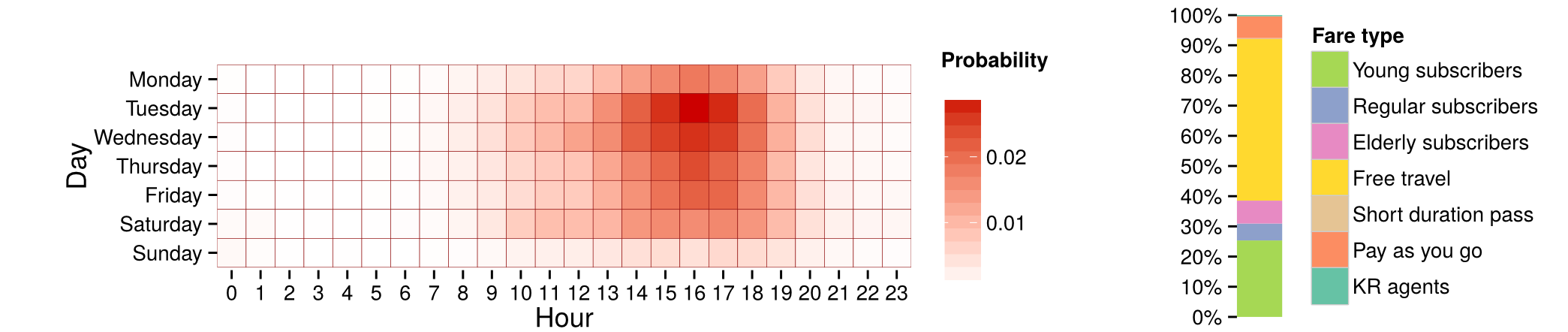Mean profile of a cluster with 8.64% of users

## Methodology

Using a continuous time description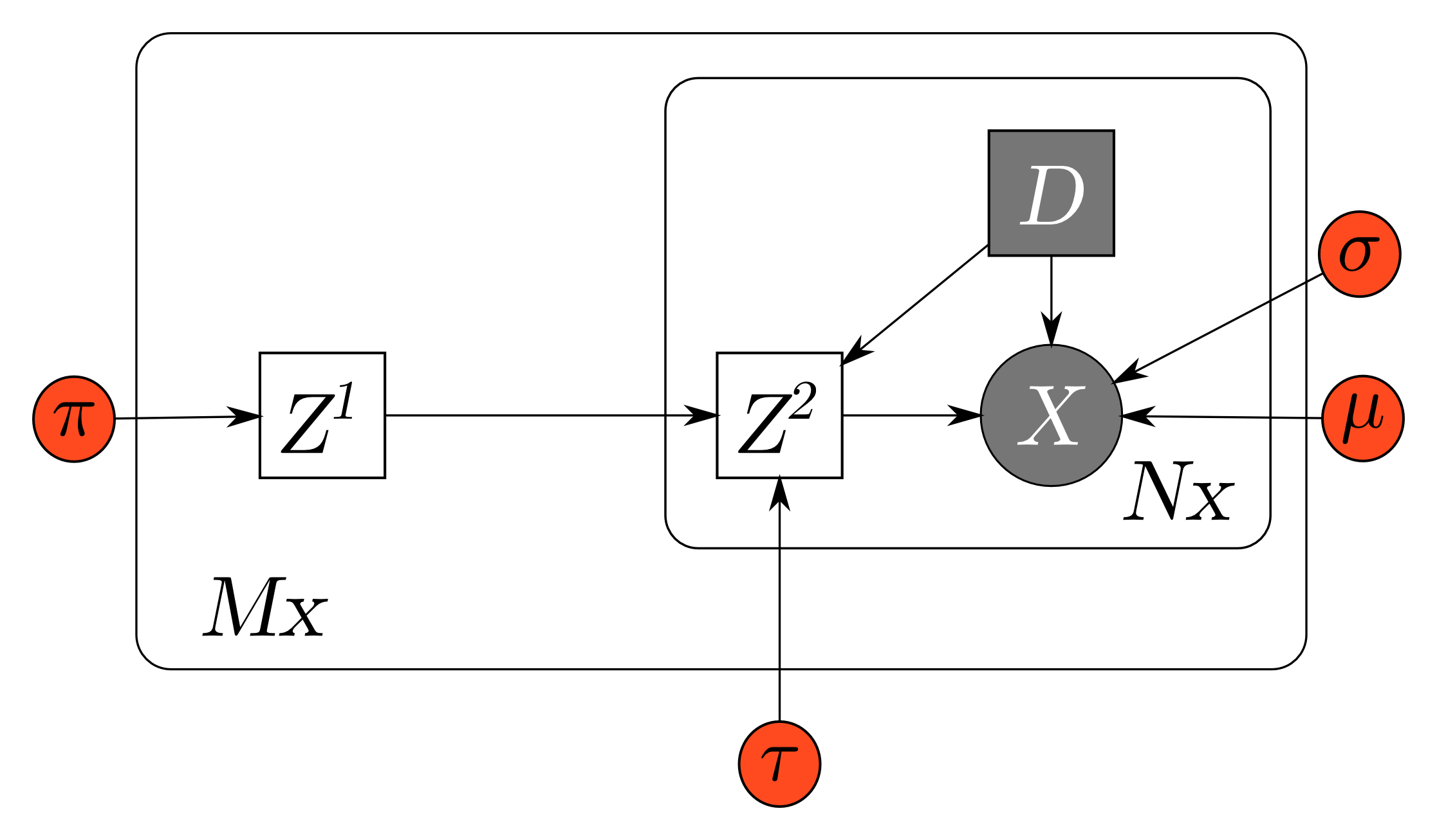Generative model for continuous time user clustering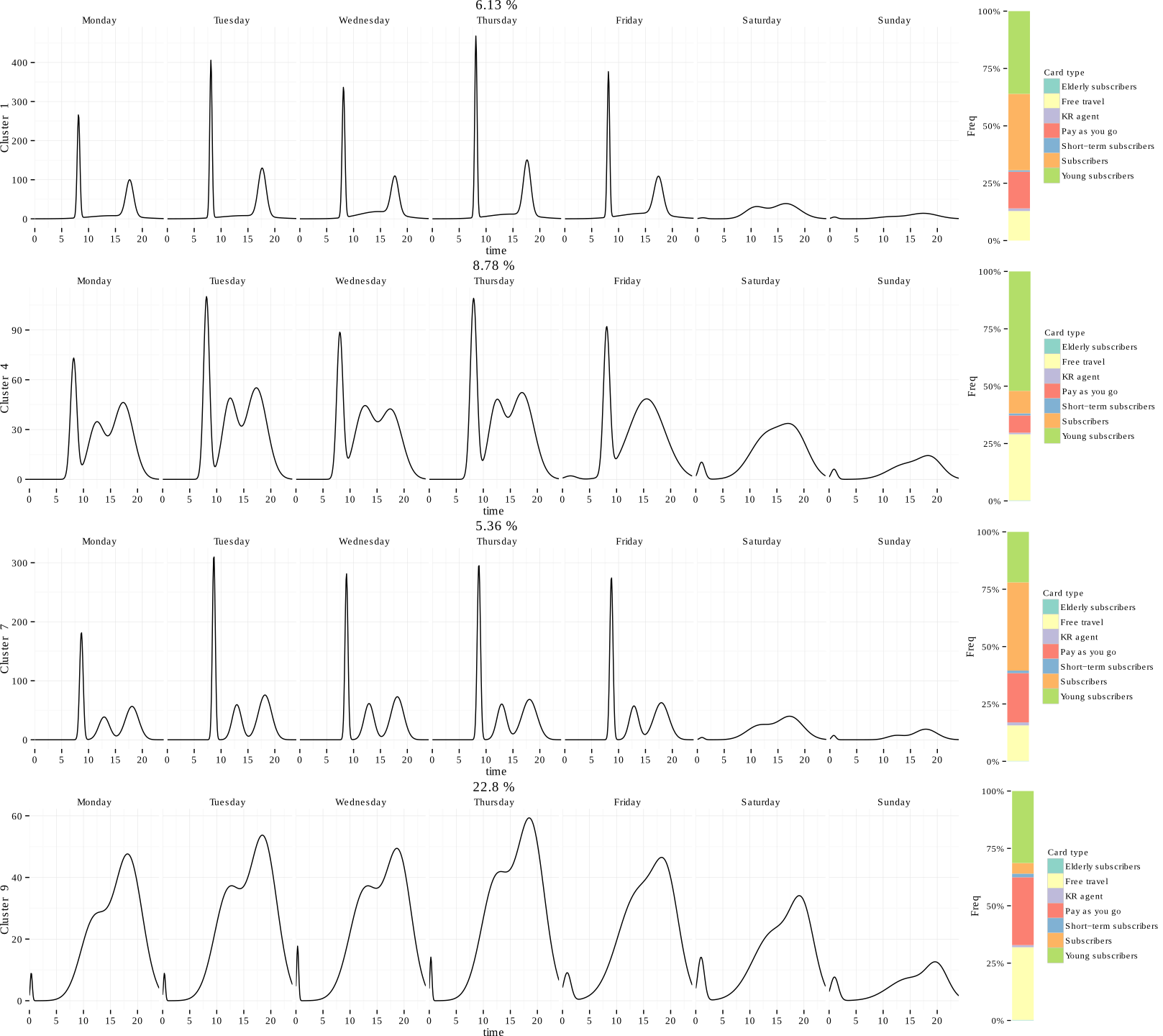# Some comments on the results

• Sub population quite different
• A quantitative view of user profile proportions
• Better understanding the user needs

# Integrated classification likelihhod

#### Model selection and bayesian regularisation for discrete latent variable models

$$ICL_{ex}(\mathbf{X},\mathbf{Z})=\int_{\mathbf{\Theta}}p(x,z;\mathbf{\theta})p(\theta)d\theta$$ $$\hat{Z}=\arg\max_{Z}ICL_{ex}(\mathbf{X},\mathbf{Z})$$
• analitical expressions with conjugate priors (SBM, dc-SBM, Gaussian Mixtures, Mixture of regressions, ...)
• penalized criterion may handle varying $K$
• algorithm : greedy, simulated annealing, genetic algortithm,...
• kill clusters in surplus, avoir loop over $K$

# Integrated classification likelihhod

#### Model selection and bayesian regularisation for discrete latent variable models

$$ICL_{ex}(\mathbf{X},\mathbf{Z})=\int_{\mathbf{\beta}}p(x|z;\mathbf{\beta})p(\beta)d\mathbf{\beta}\int_{\mathbf{\pi}}p(z|\mathbf{\pi})p(\mathbf{\pi})d\mathbf{\pi}$$ $$\pi \sim D(\alpha)$$
• greedy hierarchical algorithm to extract regularisatiojn path over $\alpha$
• parital ordering of the clusters
• dendograme and nested solutions

# Graph clustering: SBM

$$Z_i \,\sim\, \mathcal{M}(1,\pi)$$ $$X_{ij}|Z_{ik}Z_{jl}=1\,\sim\, \mathcal{B}(\beta_{kl})$$

# Graph clustering: SBM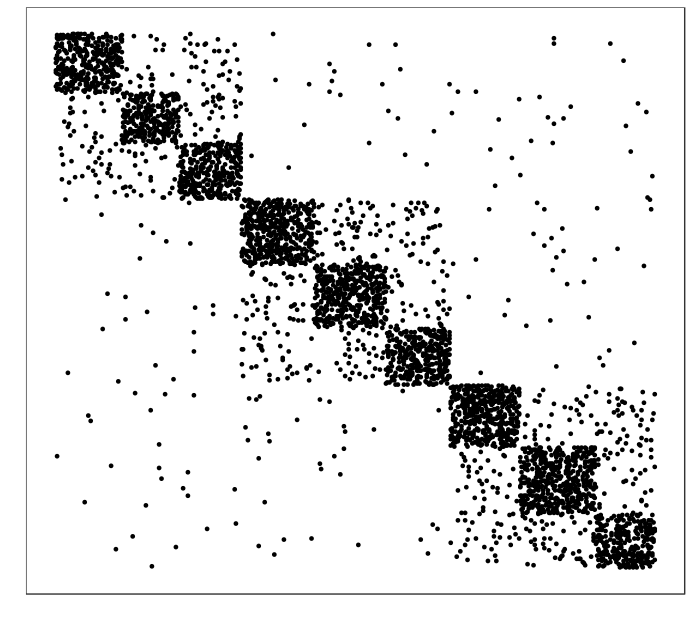# Graph clustering: SBM

#### degree correction

$$Z_i \,\sim\, \mathcal{M}(1,\pi)$$ $$X_{ij}|Z_{ik}Z_{jl}=1\,\sim\, \mathcal{P}(\theta_i\beta_{kl}\theta_j)$$

# Graph clustering: SBM

#### degree correction and oriented graphs

$$Z_i\,\sim\, \mathcal{M}(1,\pi)$$ $$X_{ij}|Z_{ik}Z_{jl}=1 \,\sim\, \mathcal{P}(\theta^{out}_i\beta_{kl}\theta^{in}_j)$$

# SBM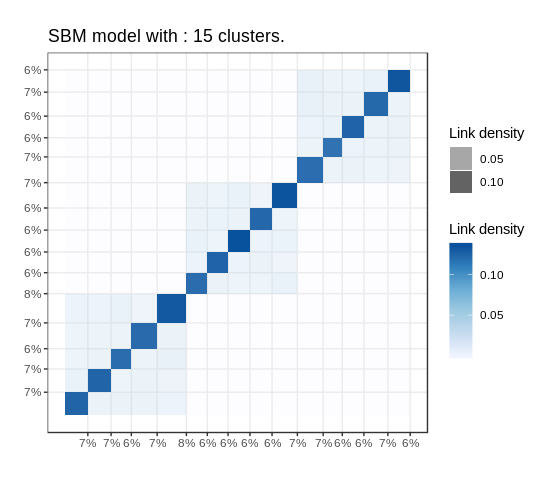simulated data

# SBM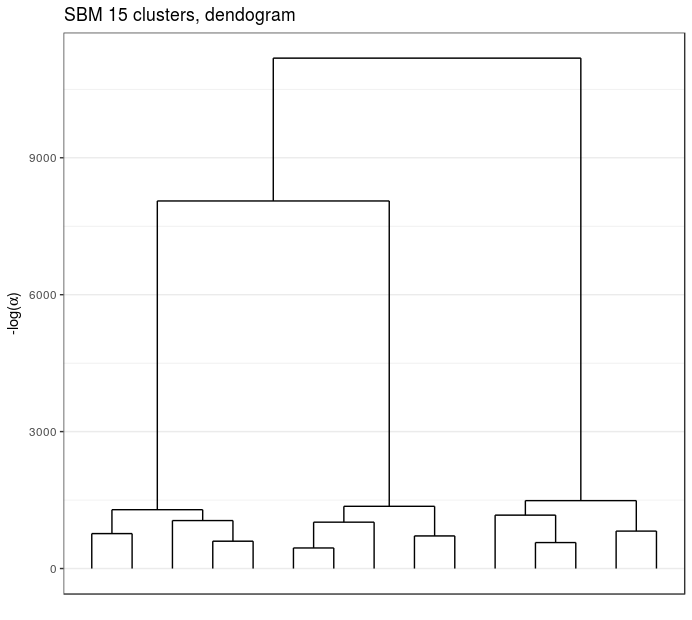simulated data

# SBM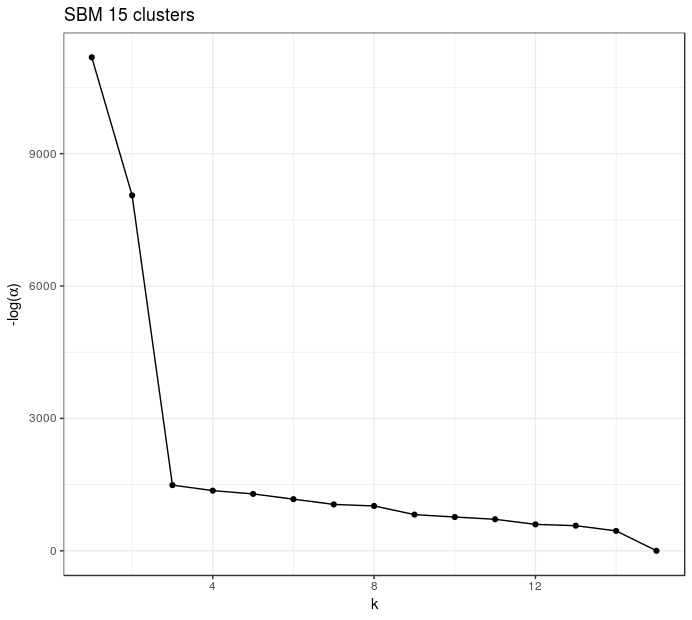simulated data

# SBM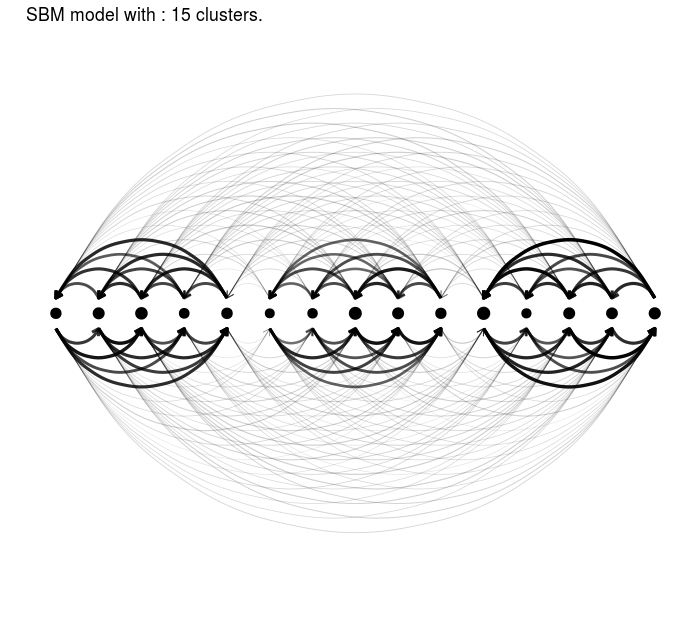simulated data

# dc-SBM, real data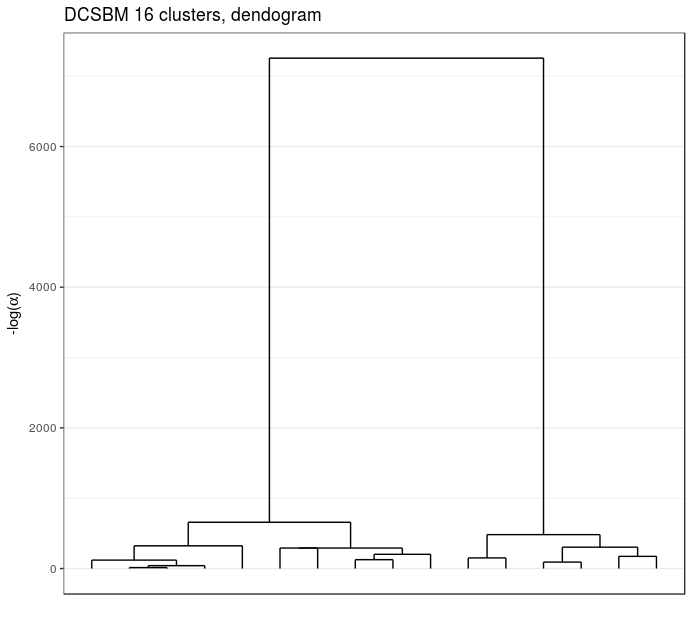Blogs politiques (US)

# dc-SBM, real data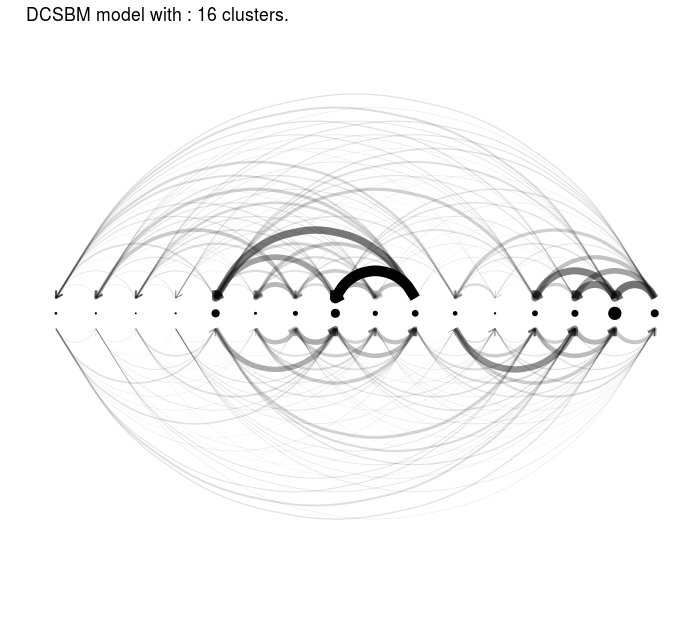Blogs politiques (US)

# dc-SBM, real data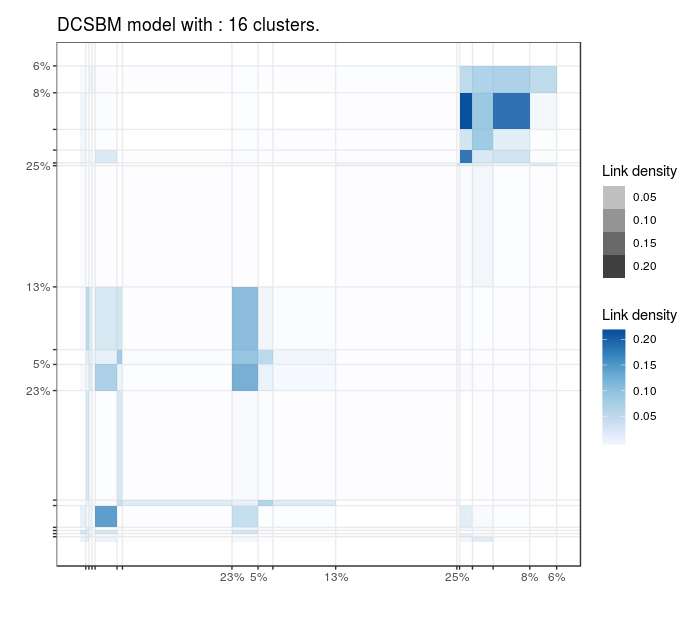Blogs politiques (US)

# dc-SBM, real data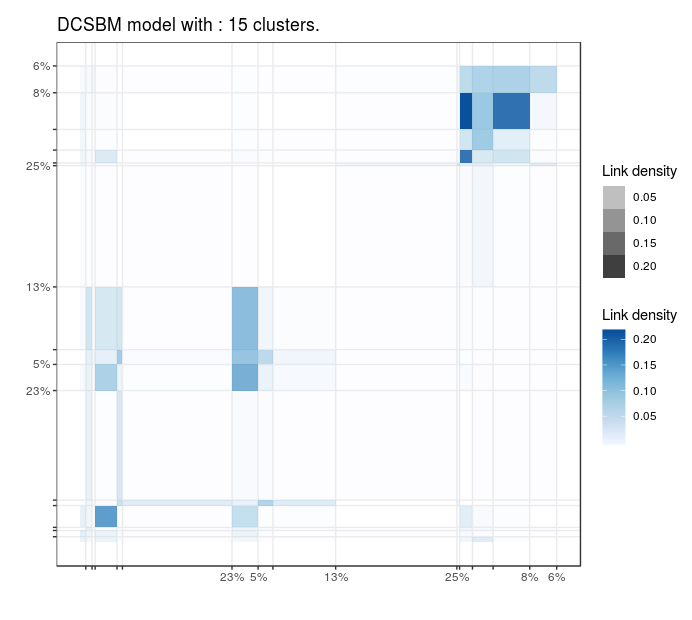Blogs politiques (US)

# dc-SBM, real data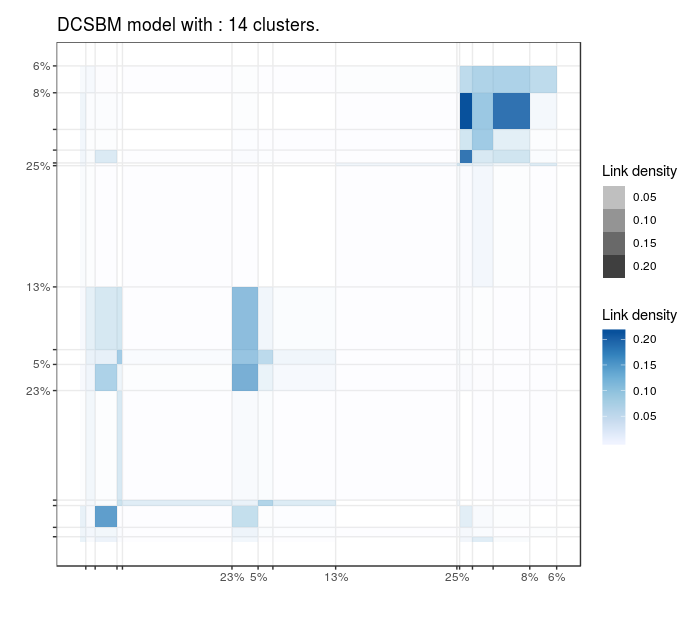Blogs politiques (US)

# dc-SBM, real data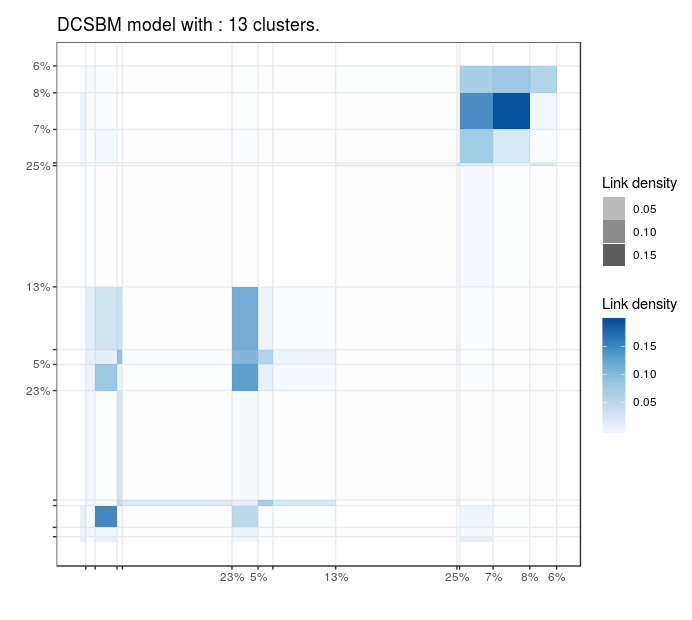Blogs politiques (US)

# dc-SBM, real dataBlogs politiques (US)

# dc-SBM, real data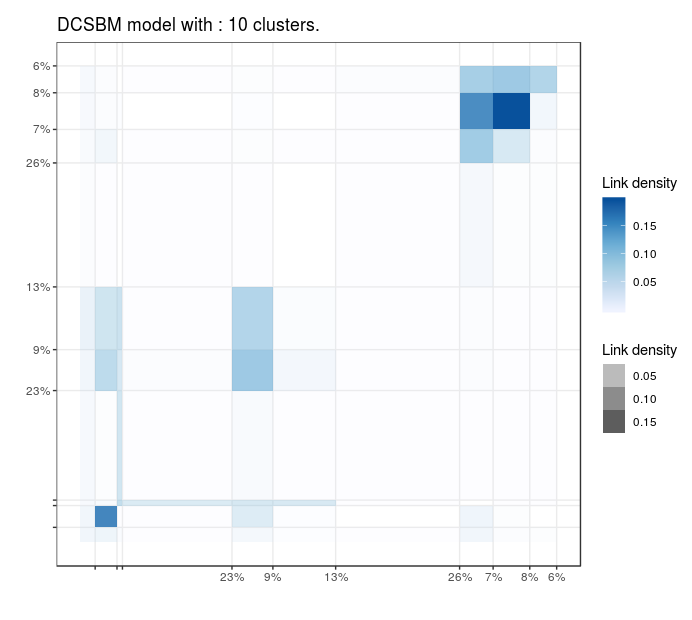Blogs politiques (US)

# dc-SBM, real data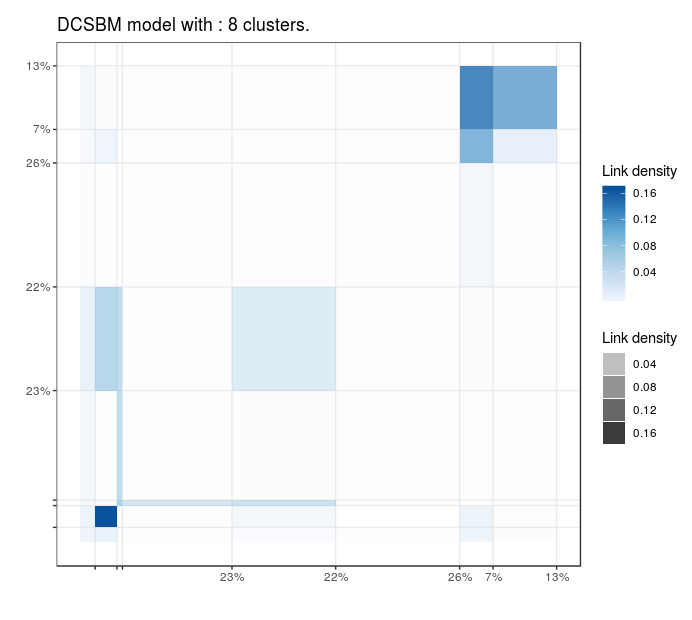Blogs politiques (US)

# dc-SBM, real data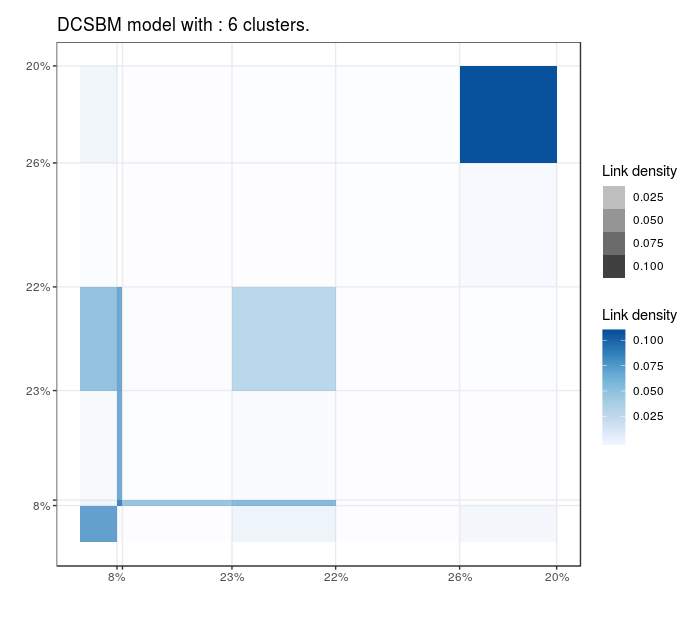Blogs politiques (US)

# dc-SBM, real data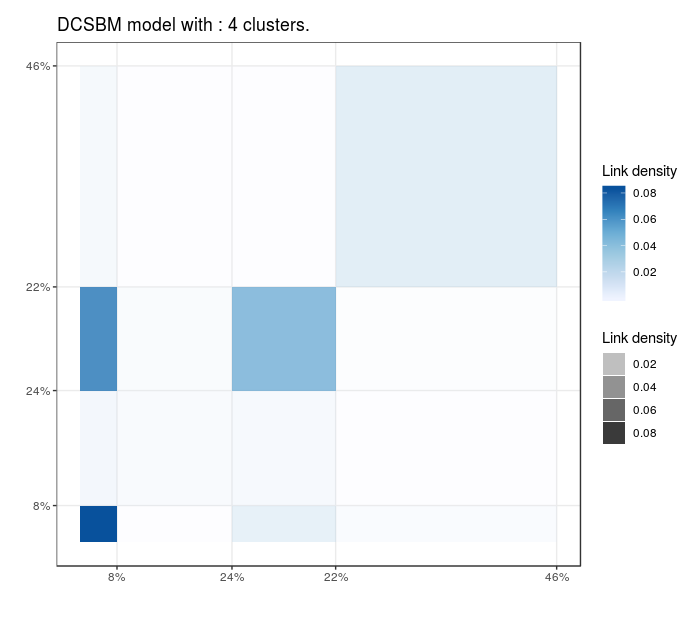Blogs politiques (US)

# dc-SBM, real data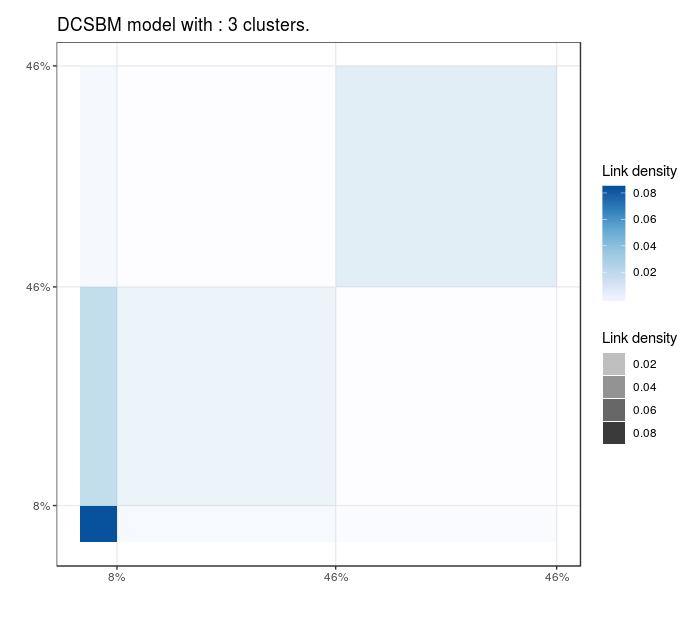Blogs politiques (US)

# dc-SBM, real data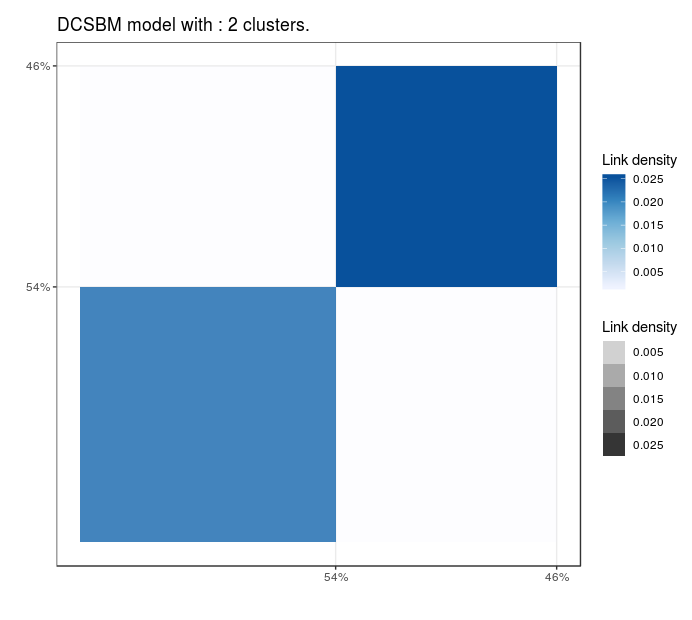Blogs politiques (US)

#### Conclusion

• Smart card data are rich and enable a analysis of spatial and temporal variability of the demand
• Exploratory analysis to highlight these variations
• User's id are interesting to reconstruct destination and

#### Current works

• Model selection and bayesian regularisation for discrete latent variable models
• Extension of gravity models // dc-SBM
• Graph embedding
• Anomaly detection and characterization
• Work on larger networks
• Network of users interactions